Successfully reported this slideshow.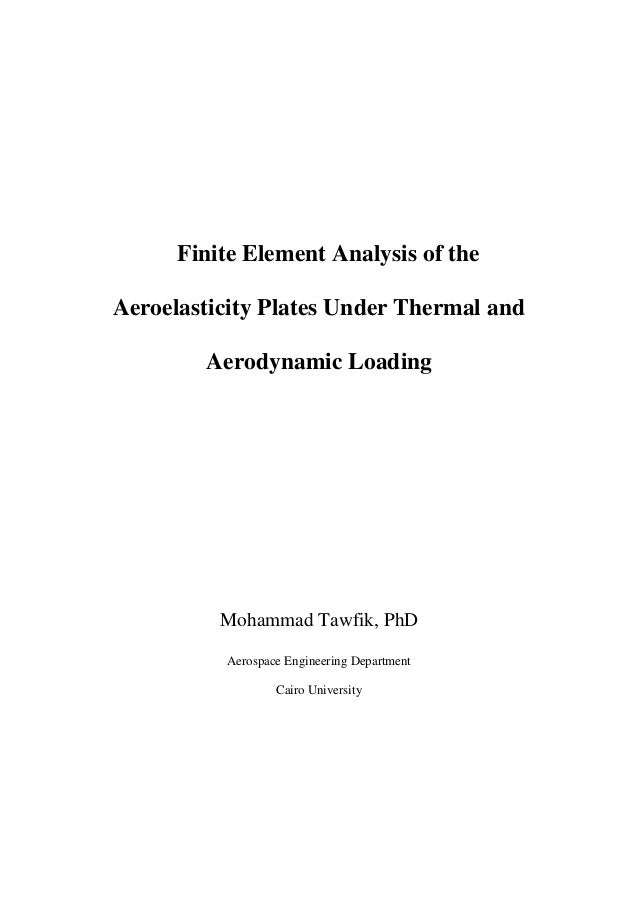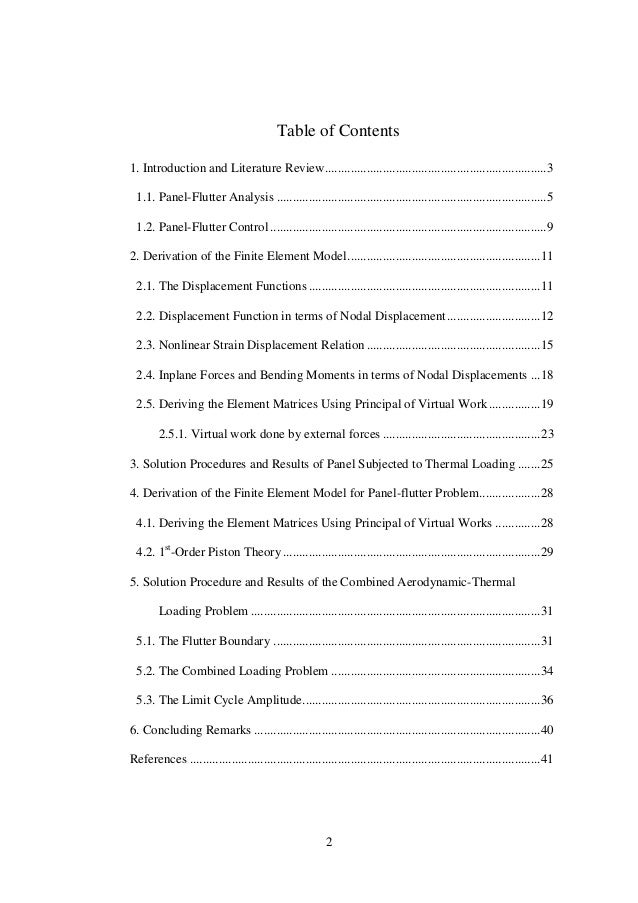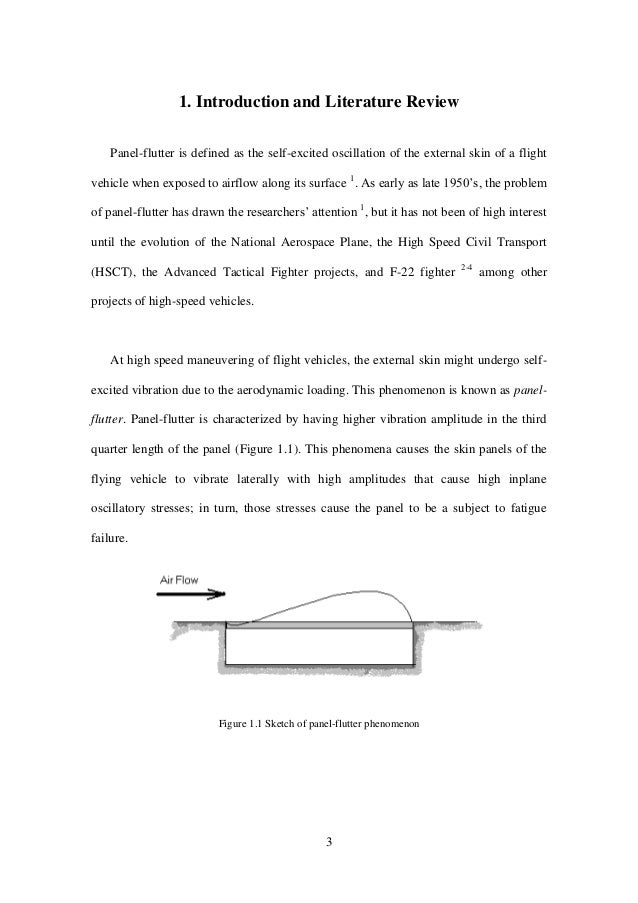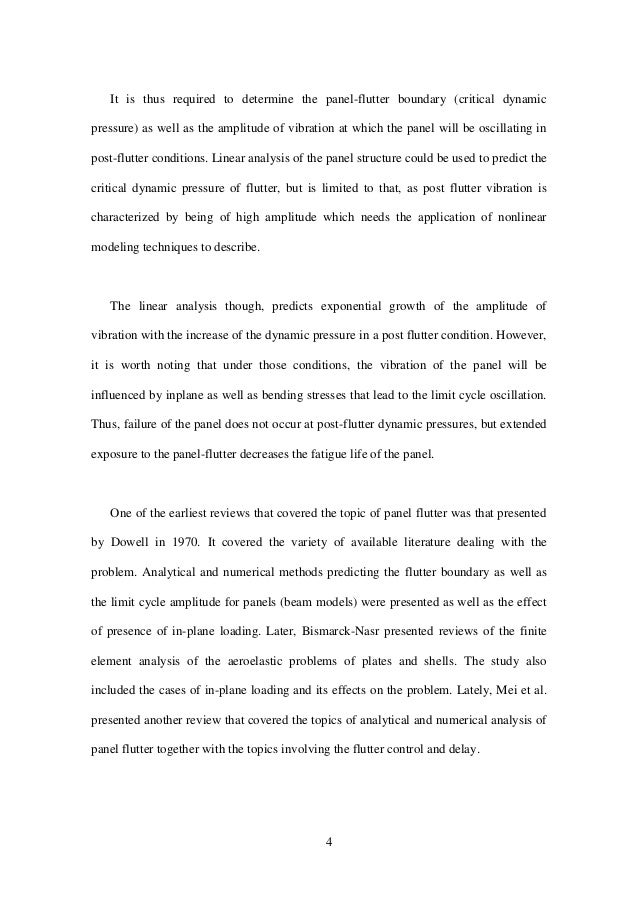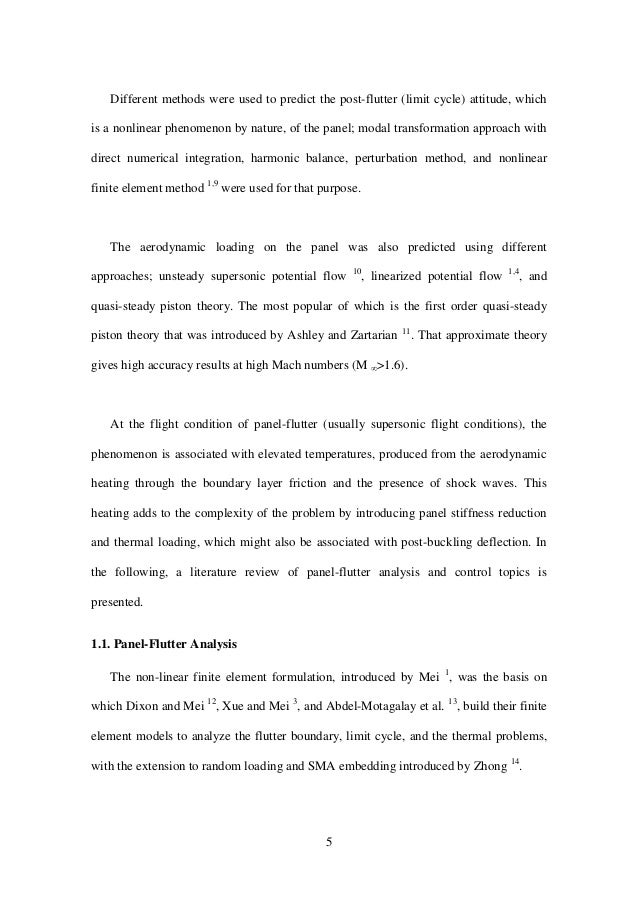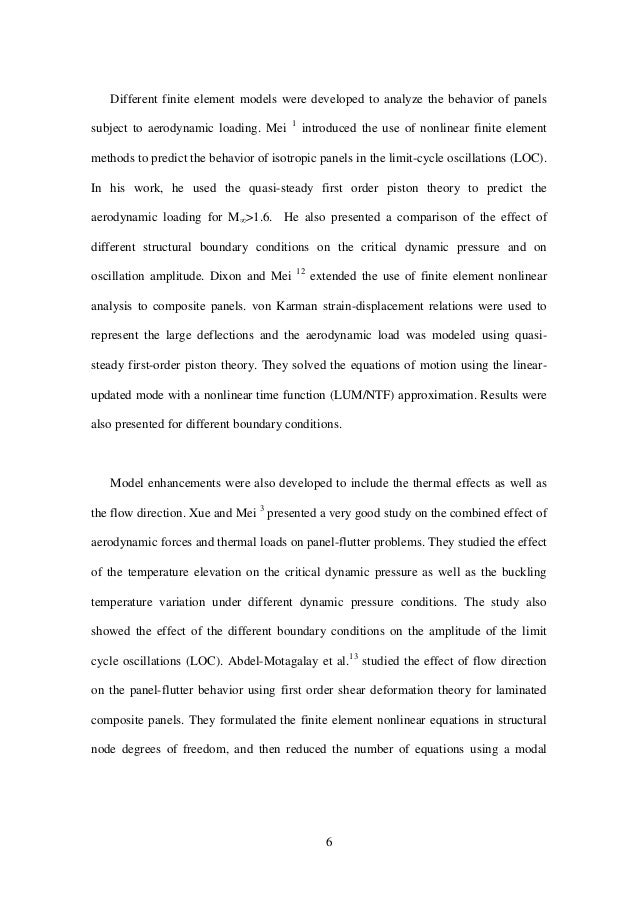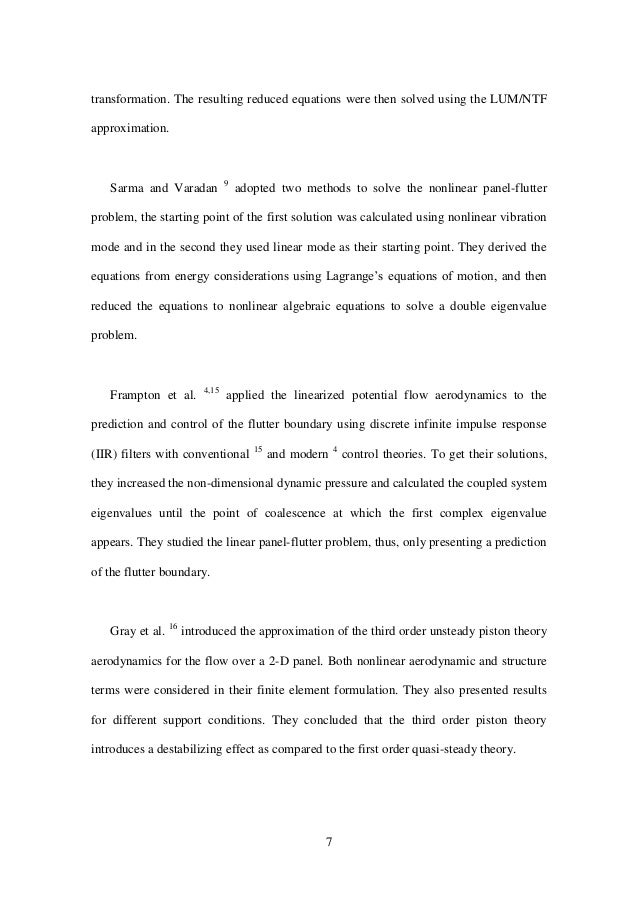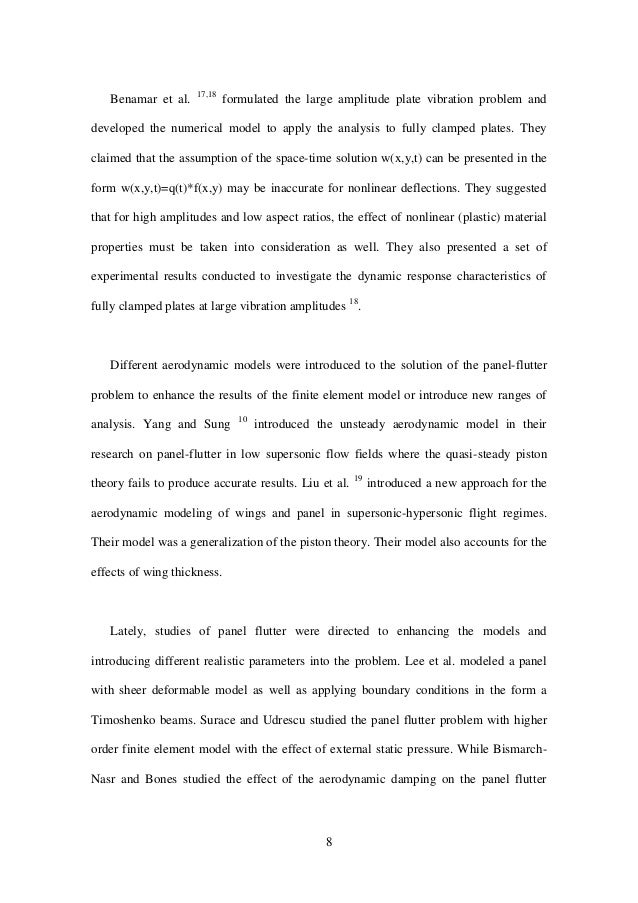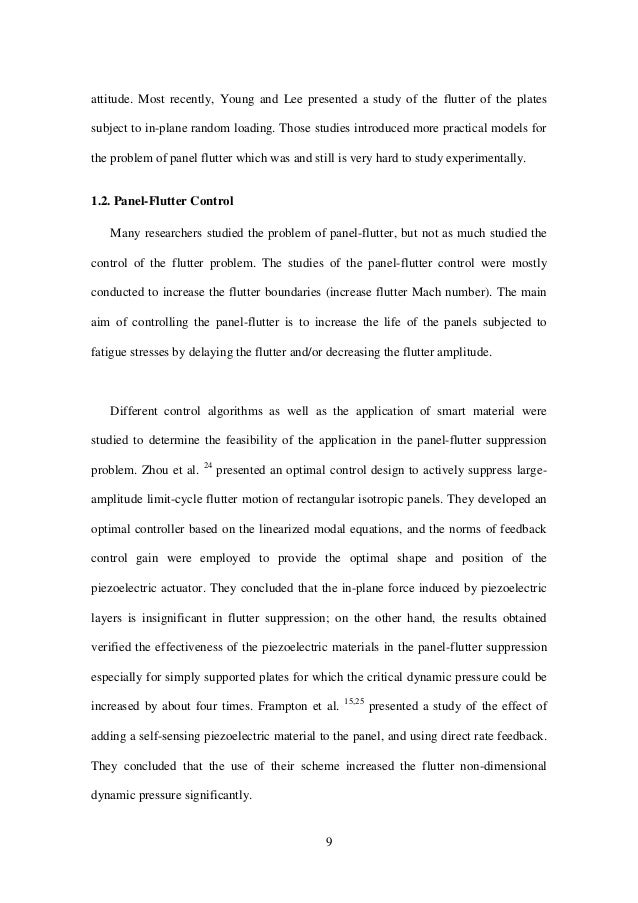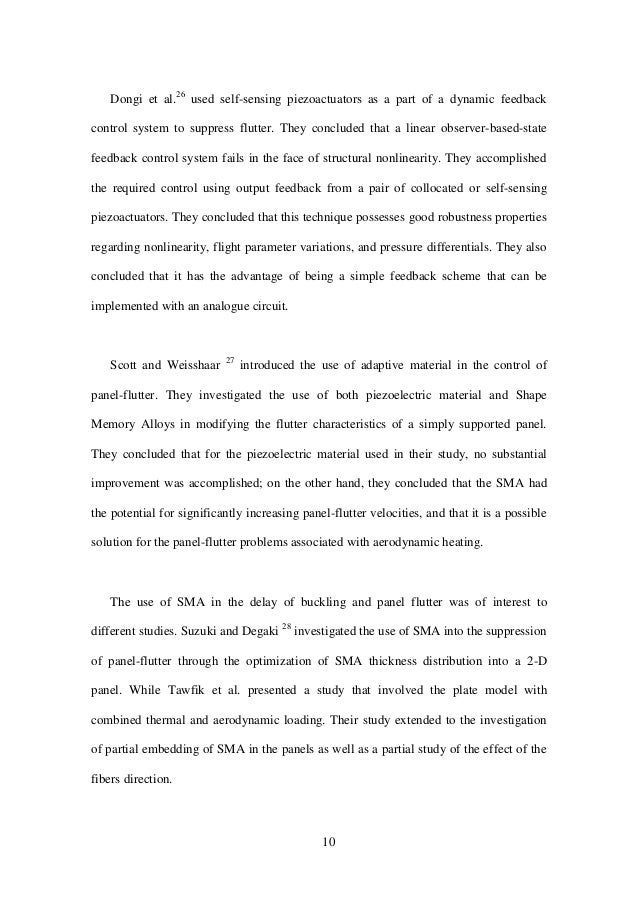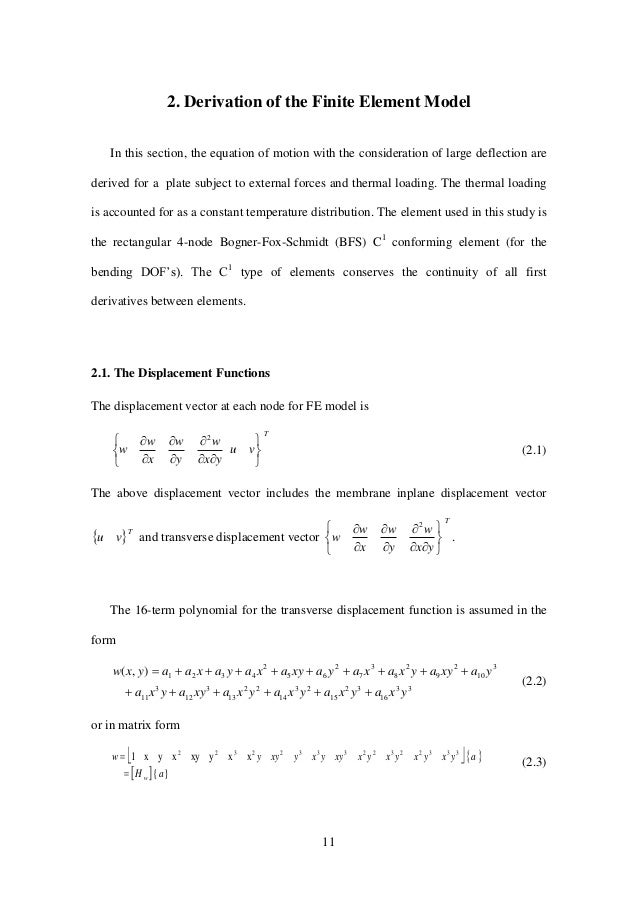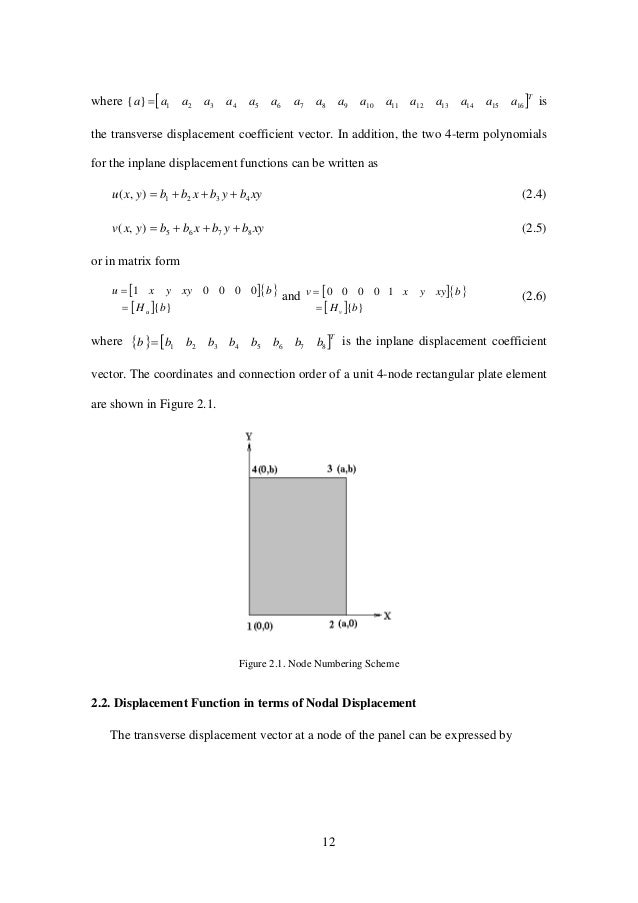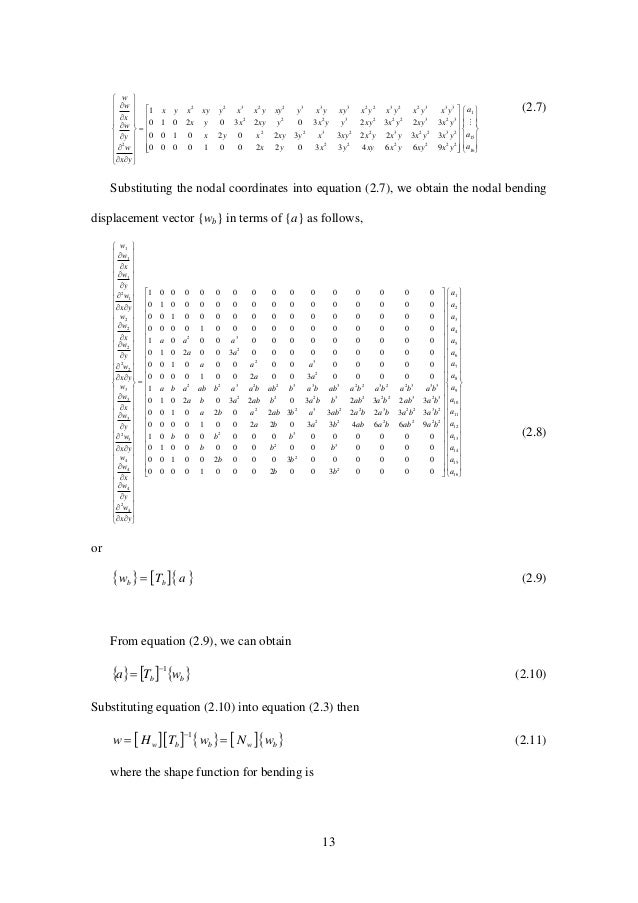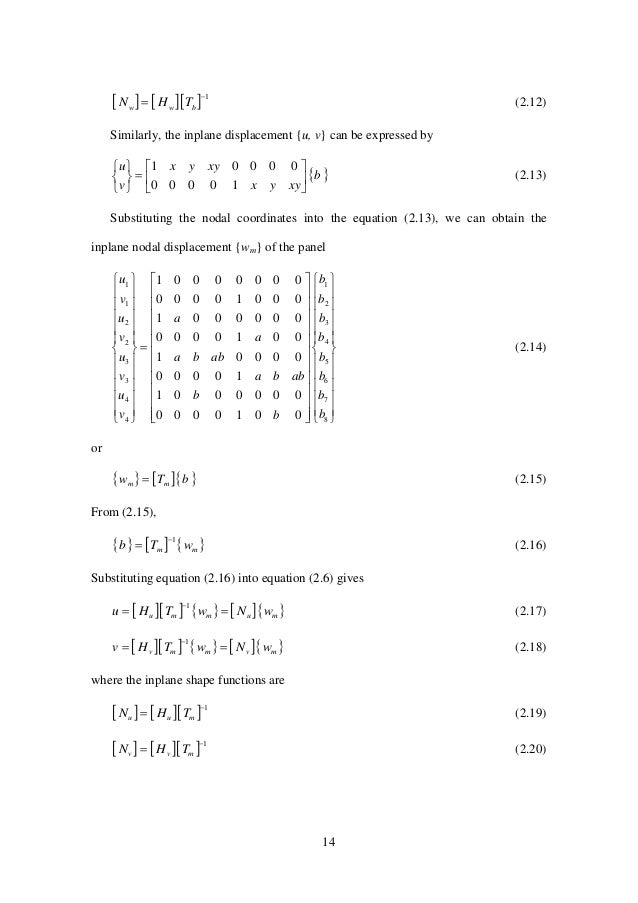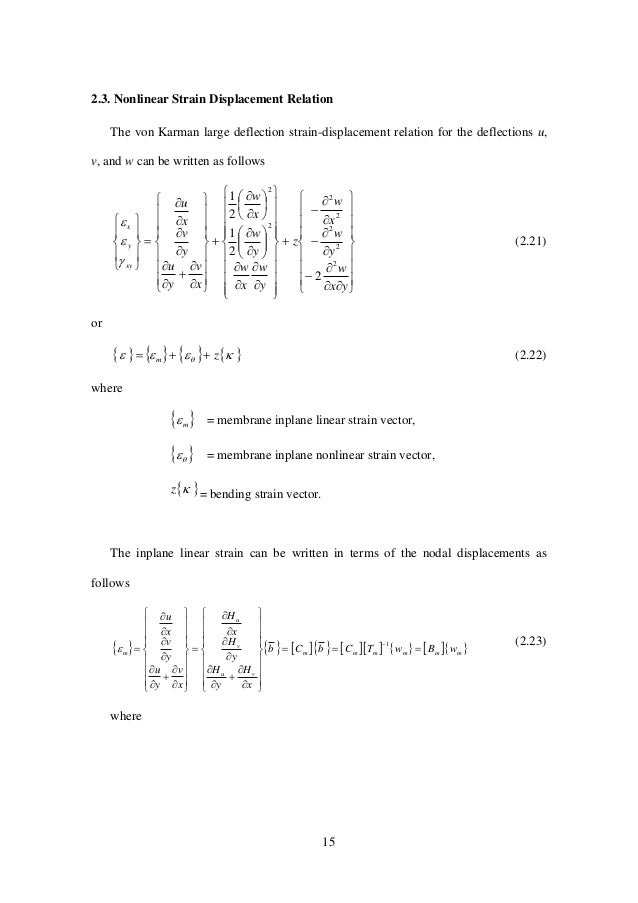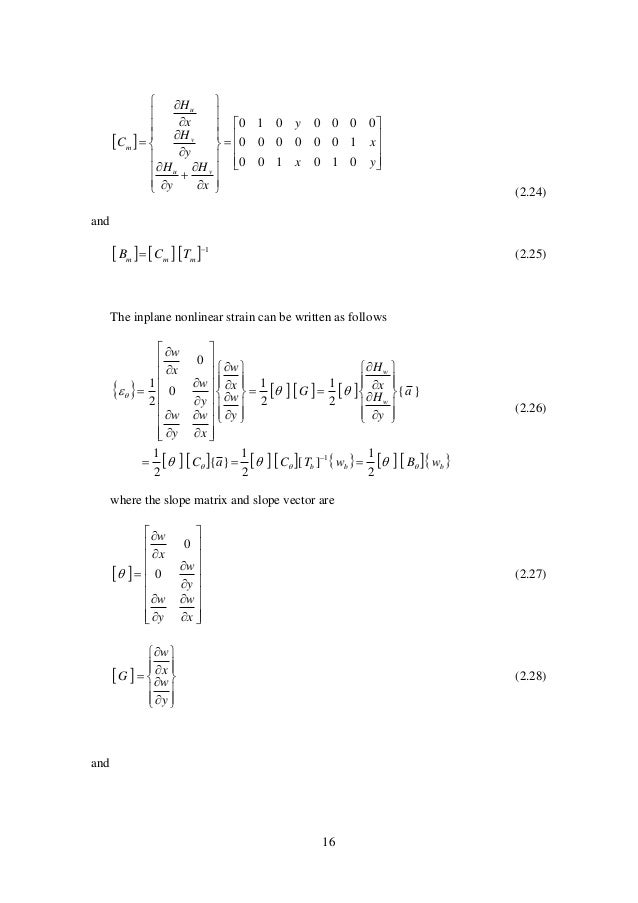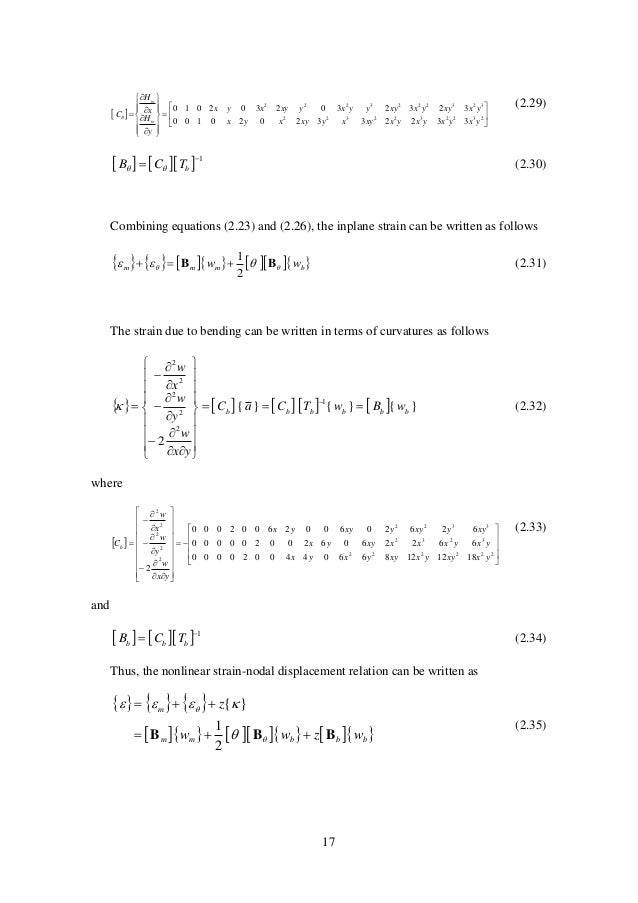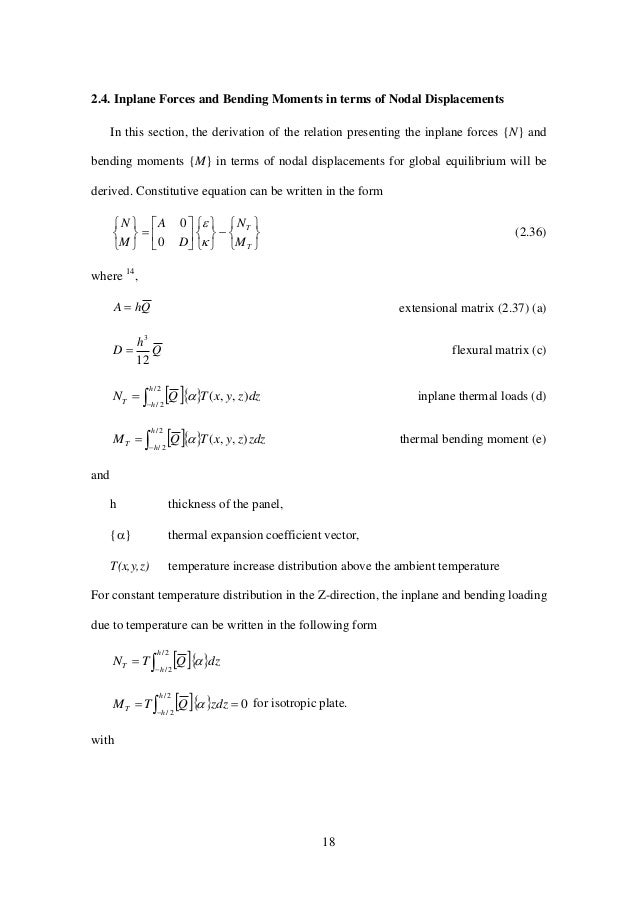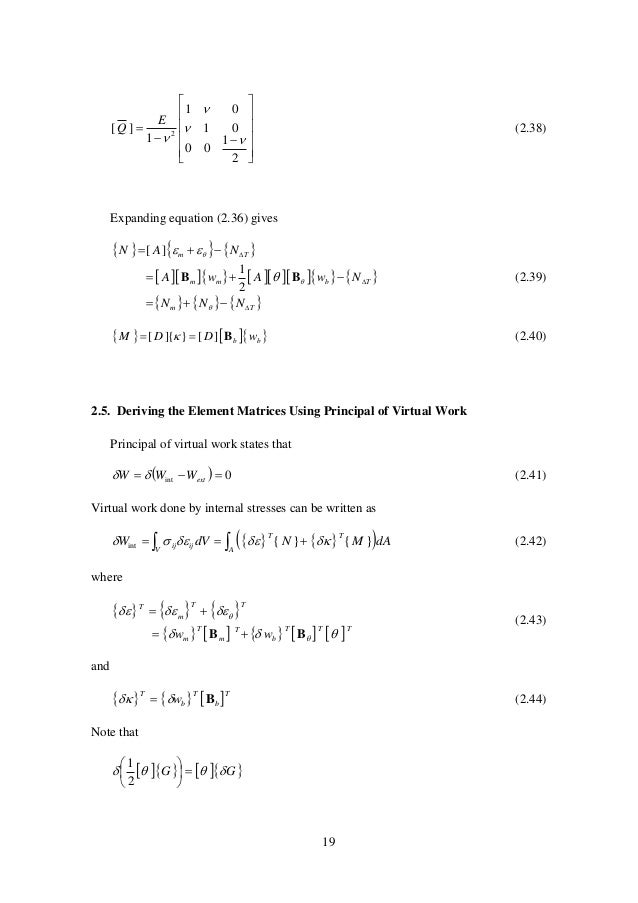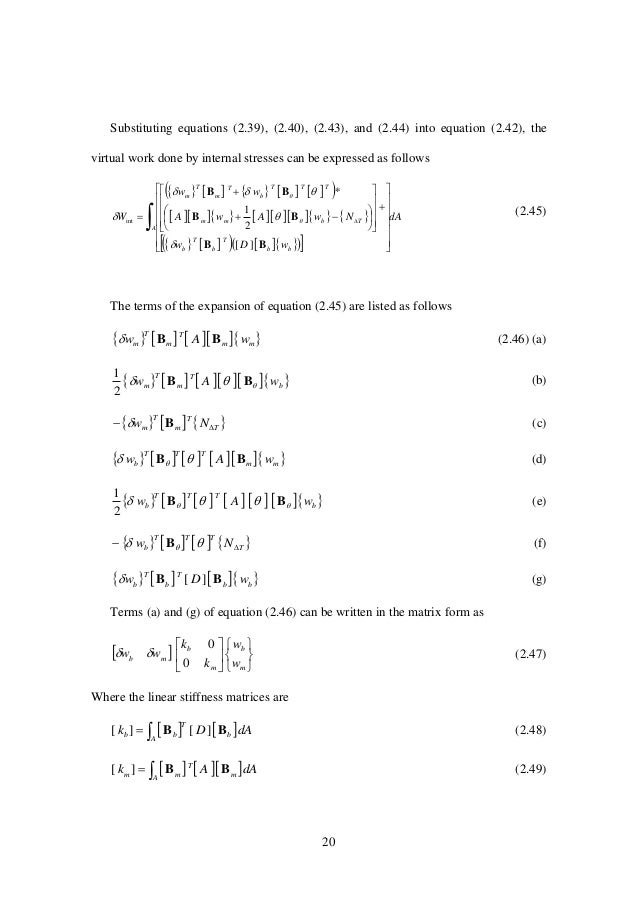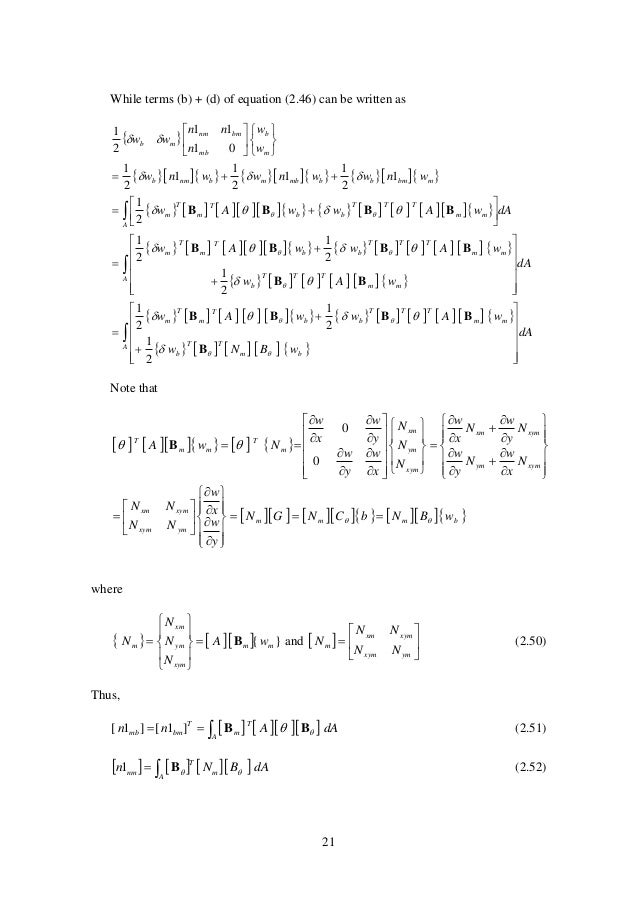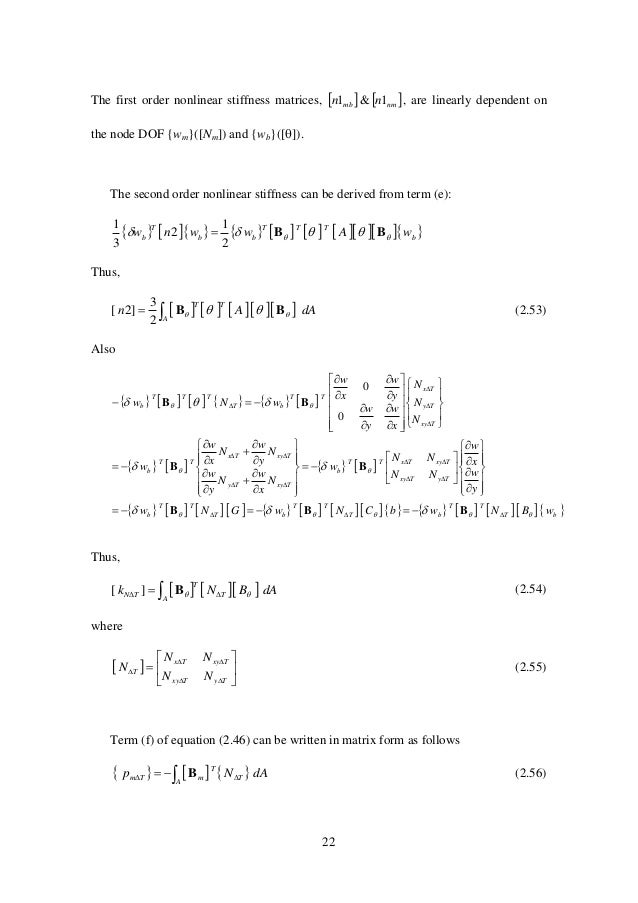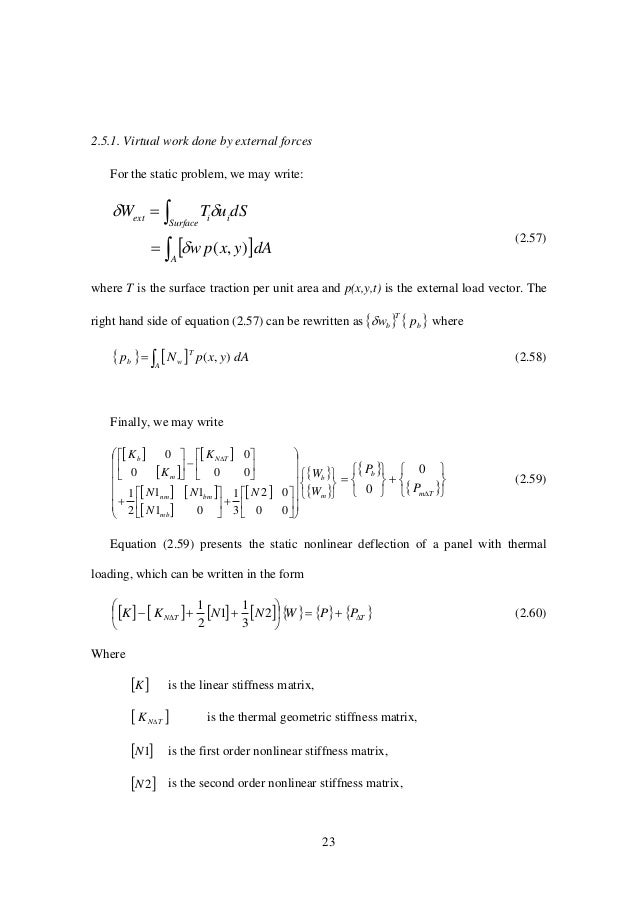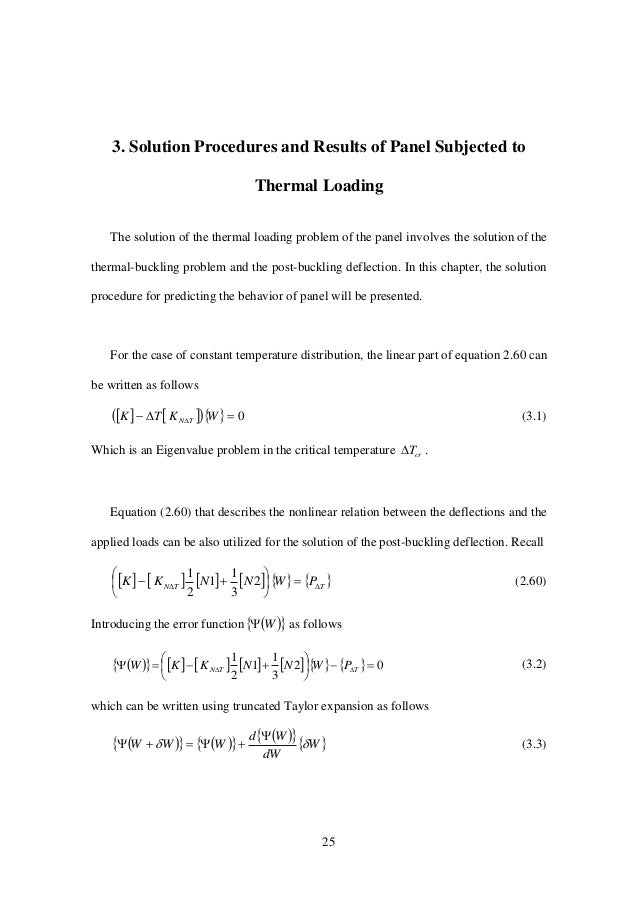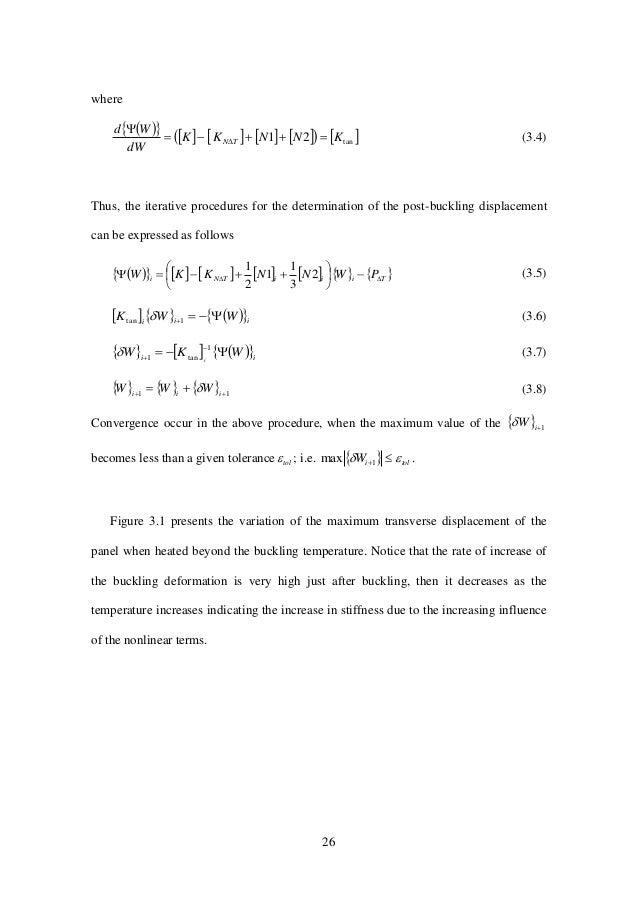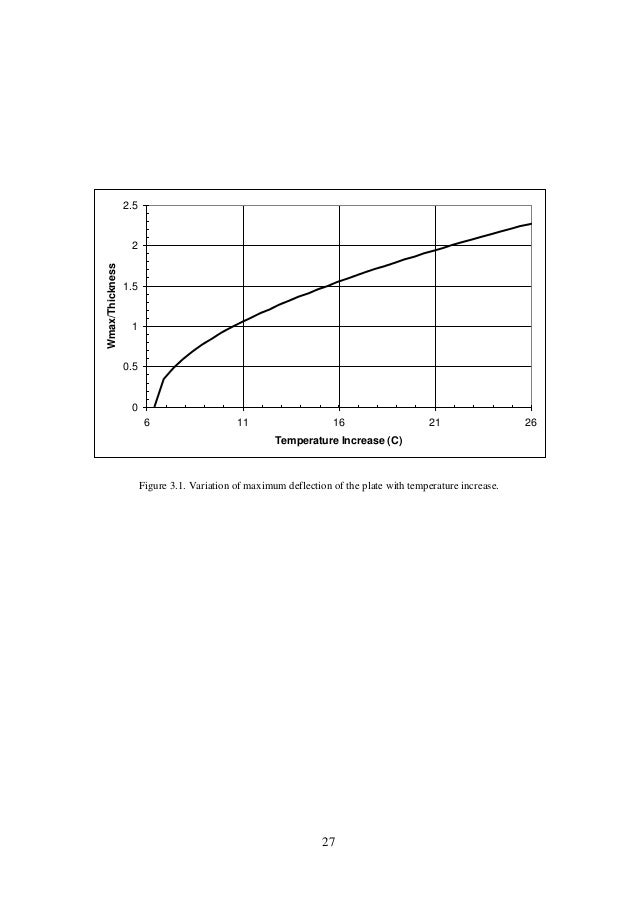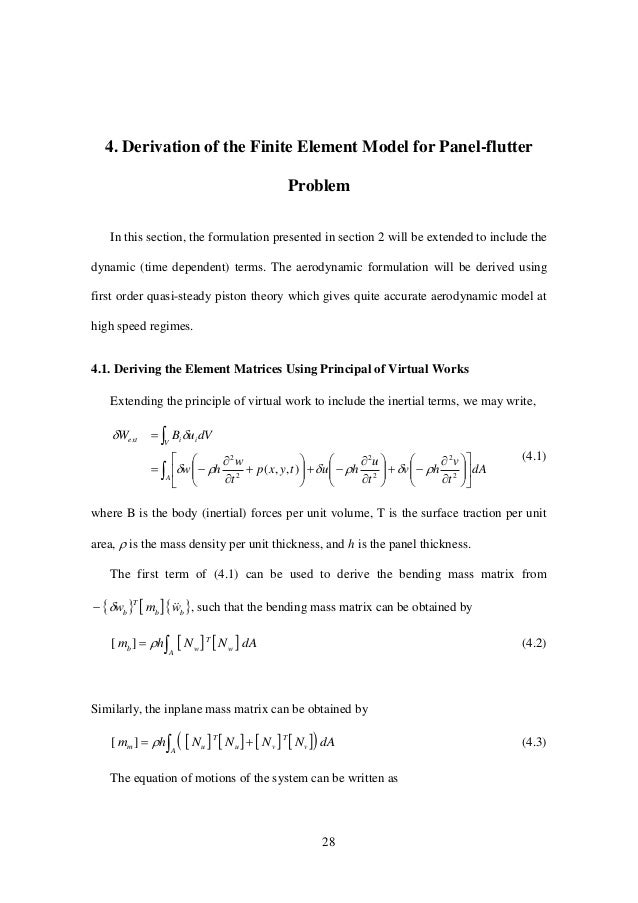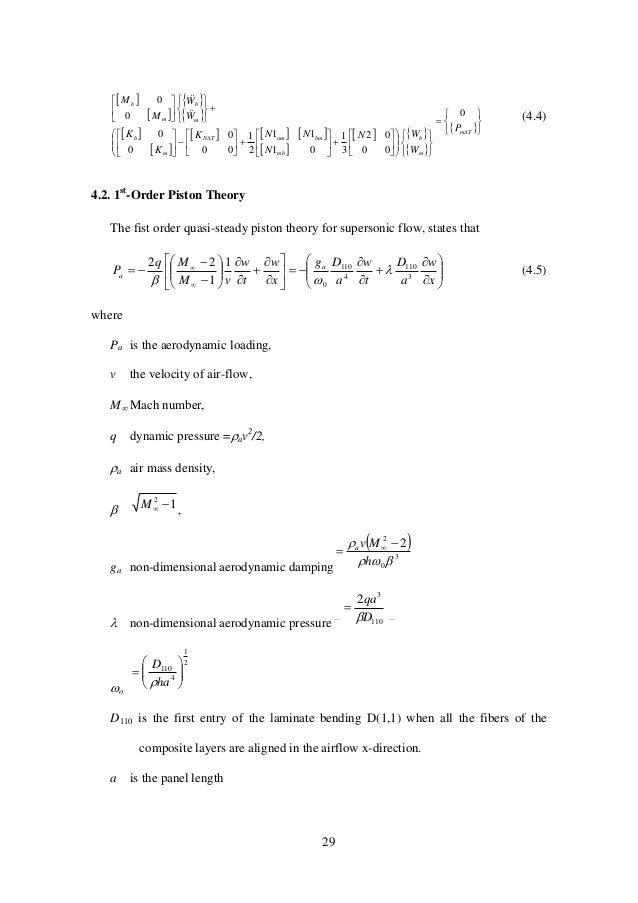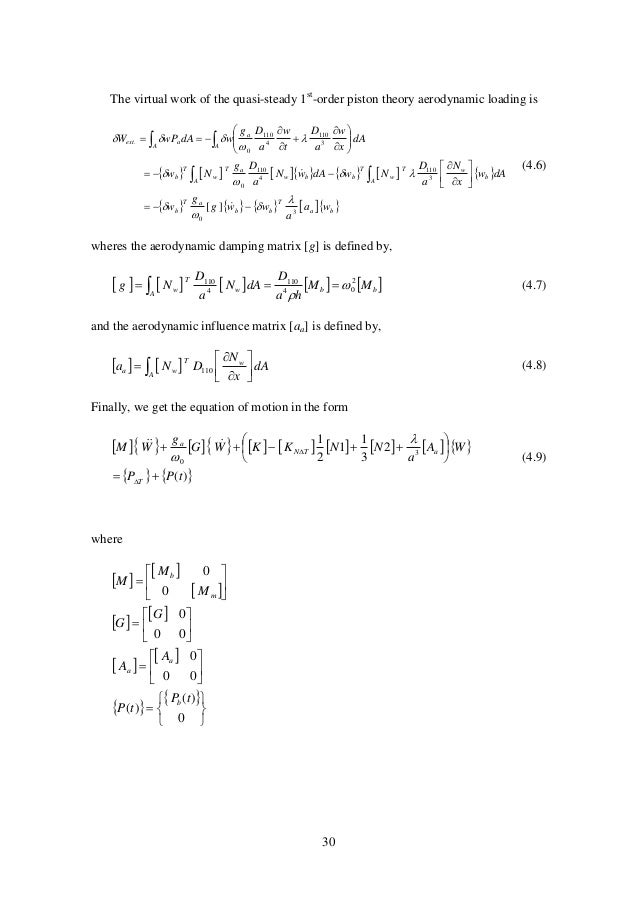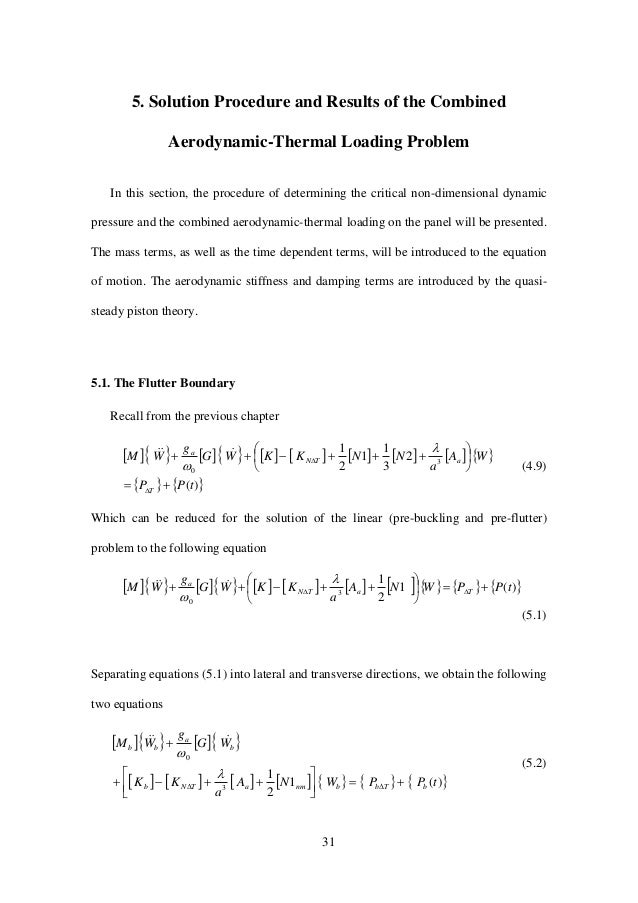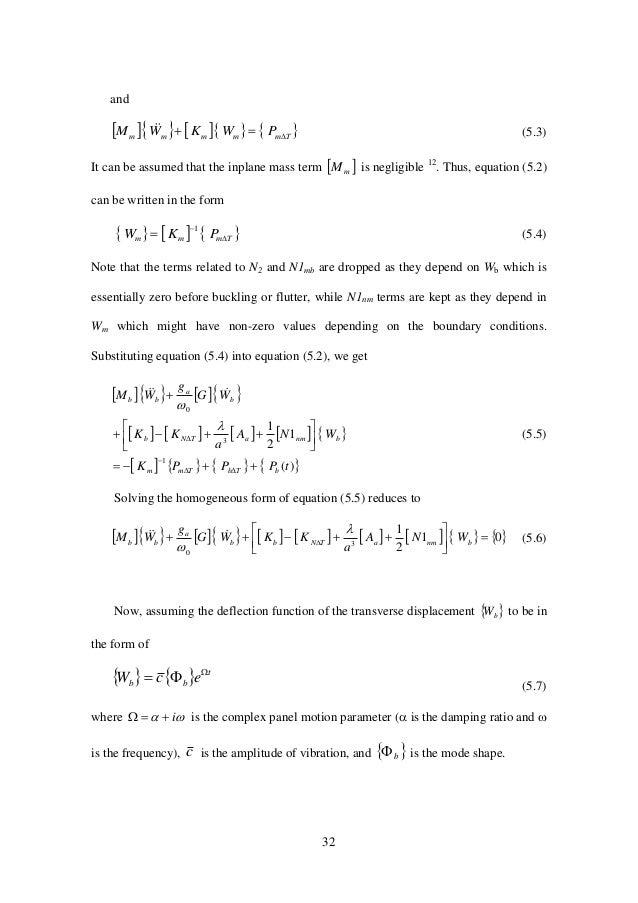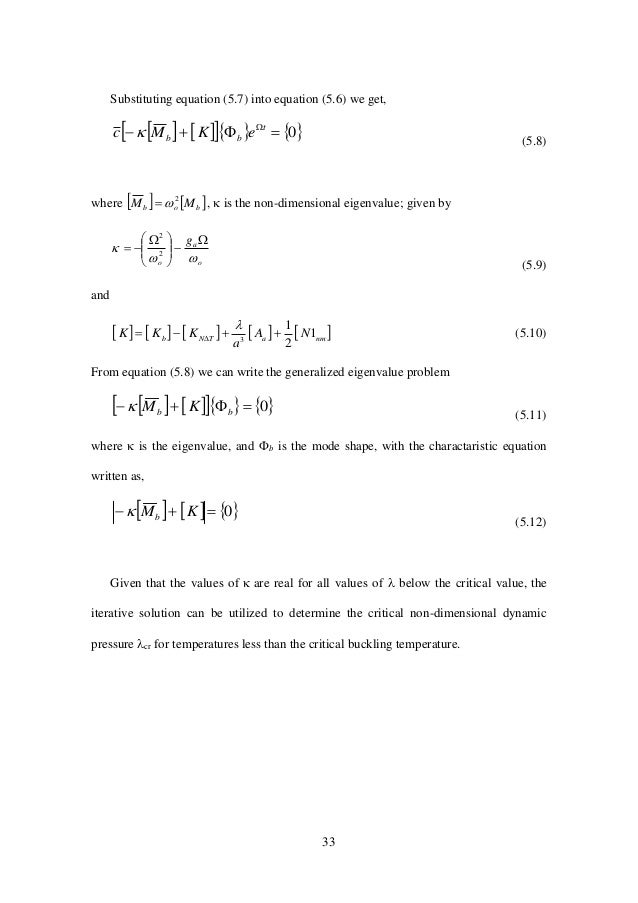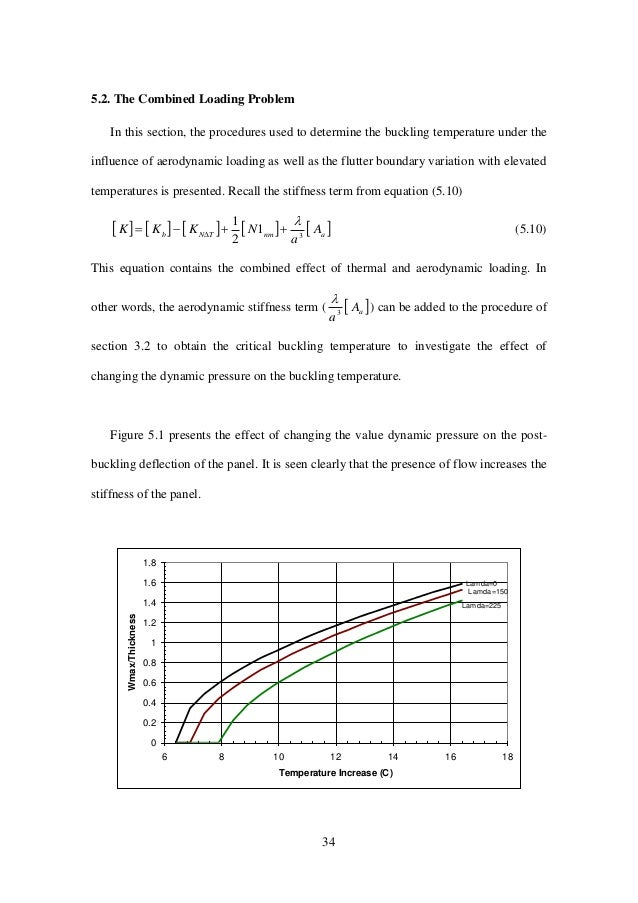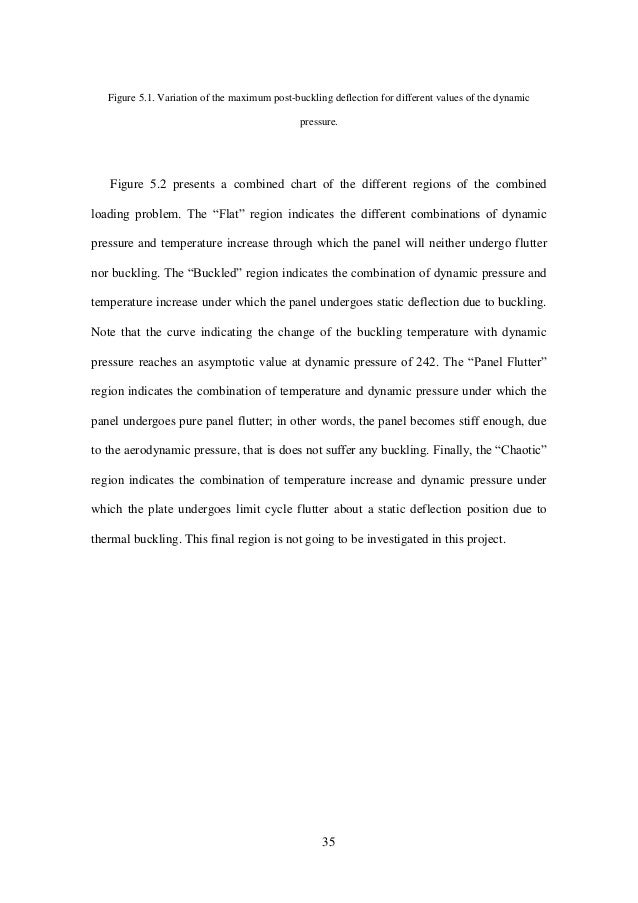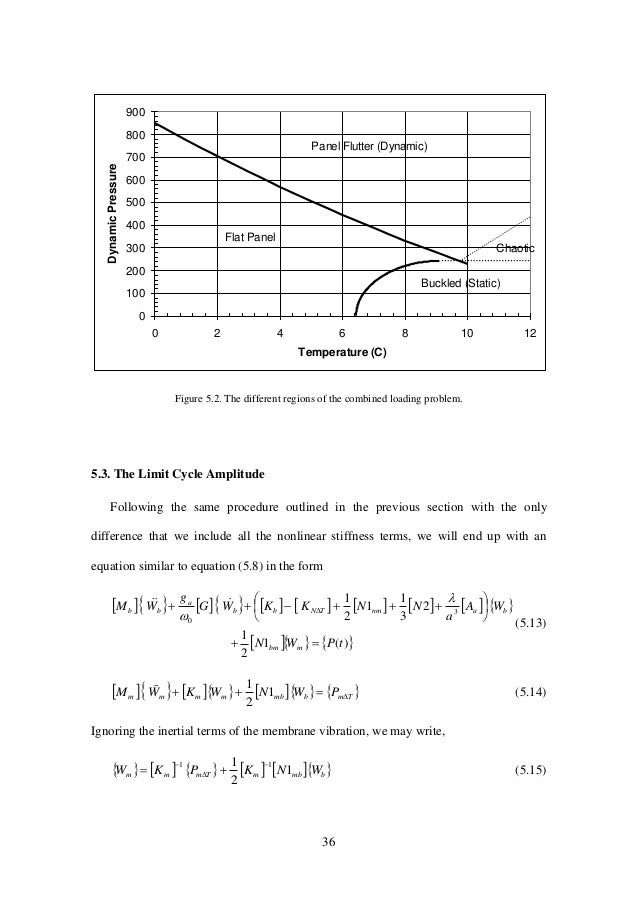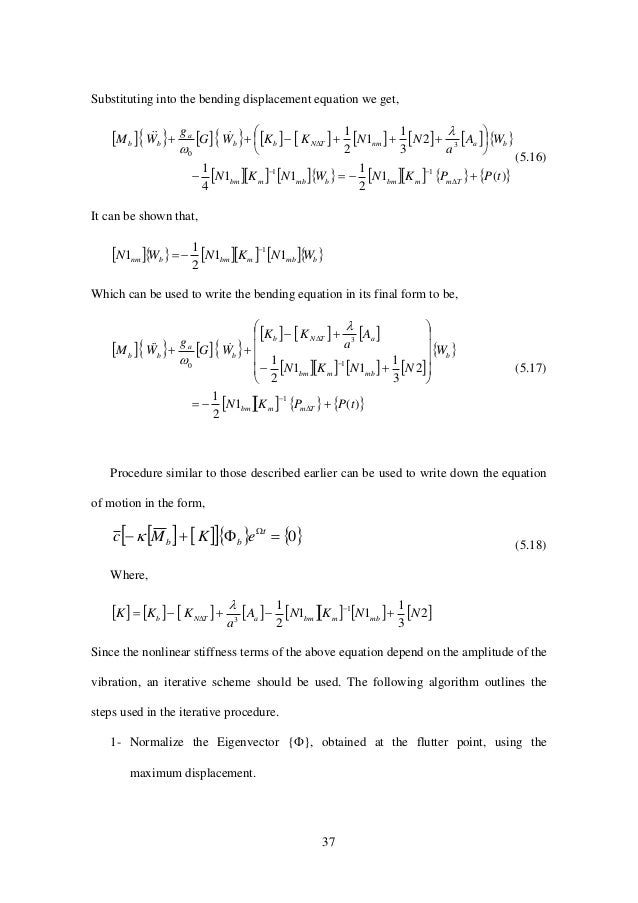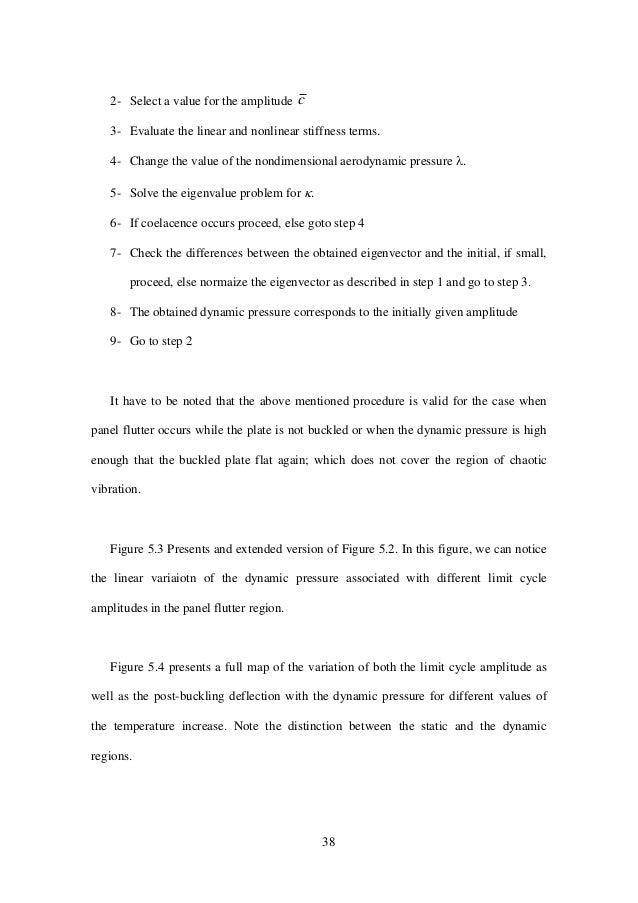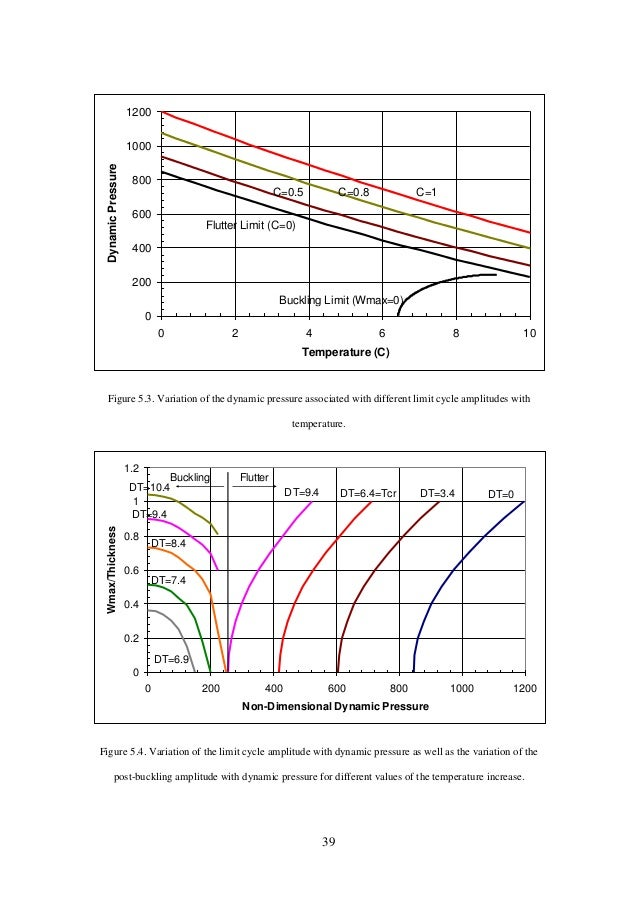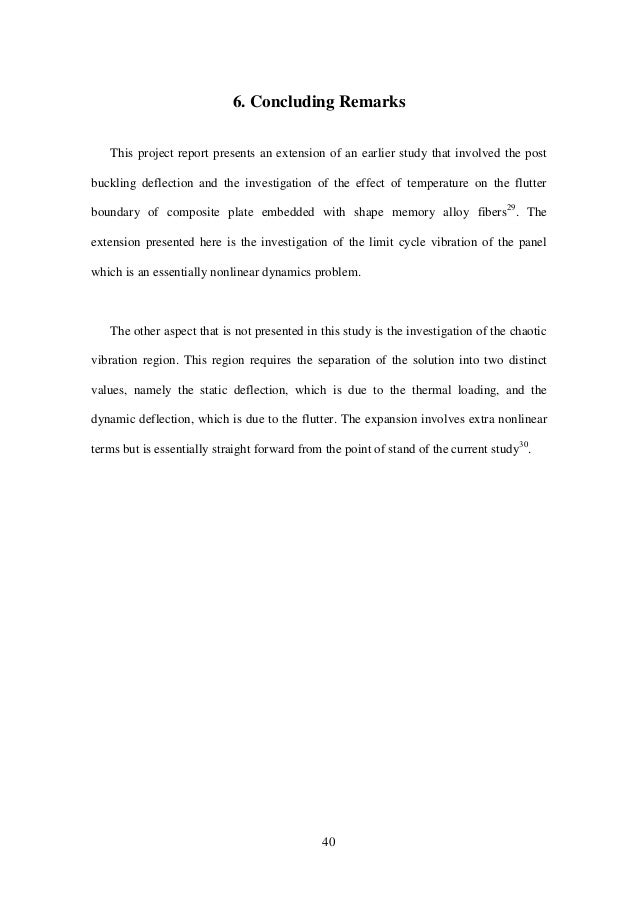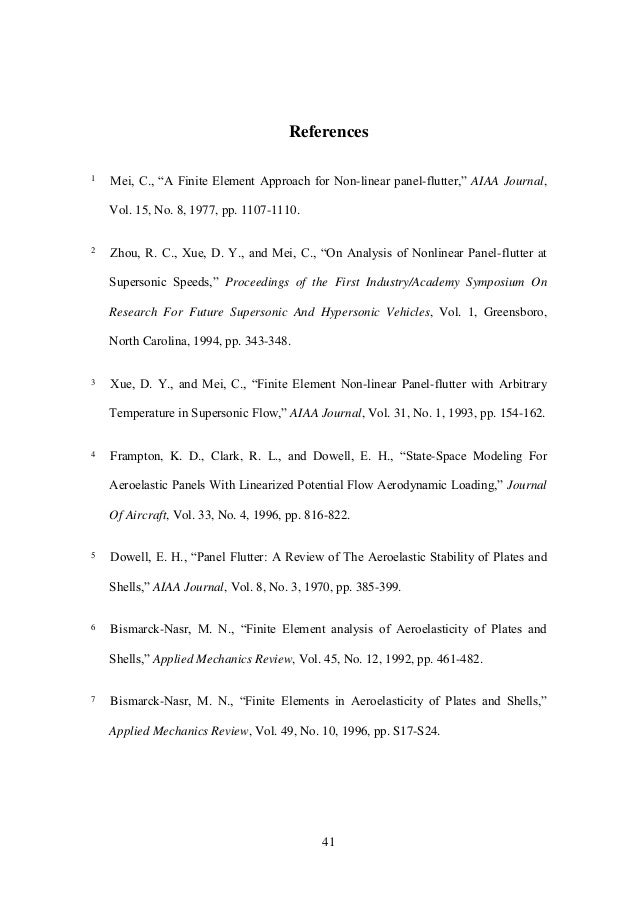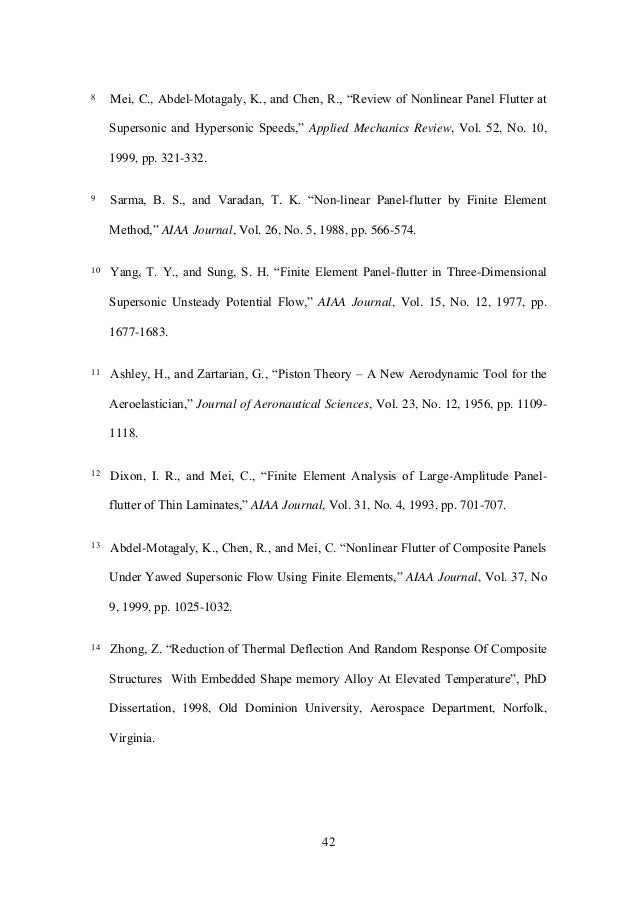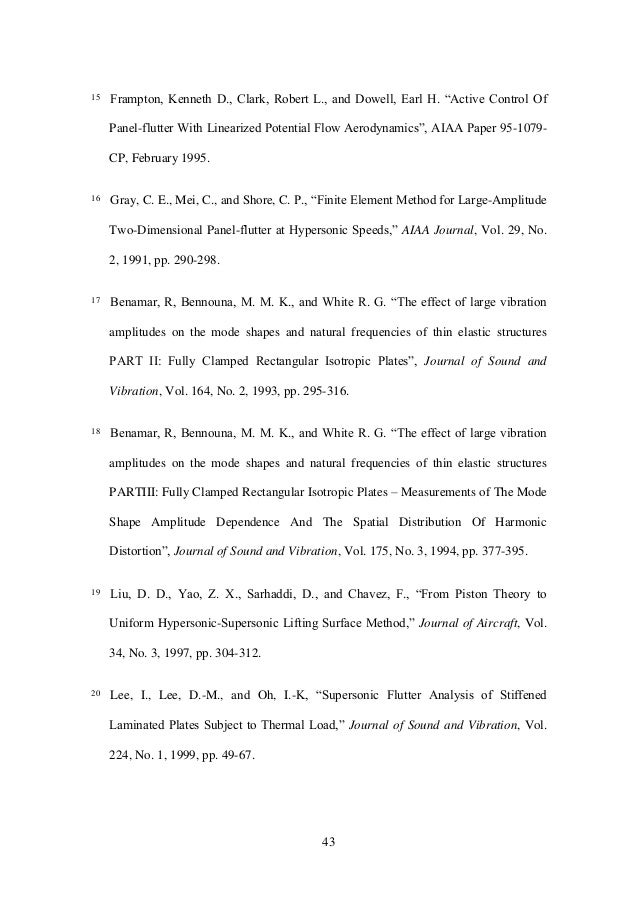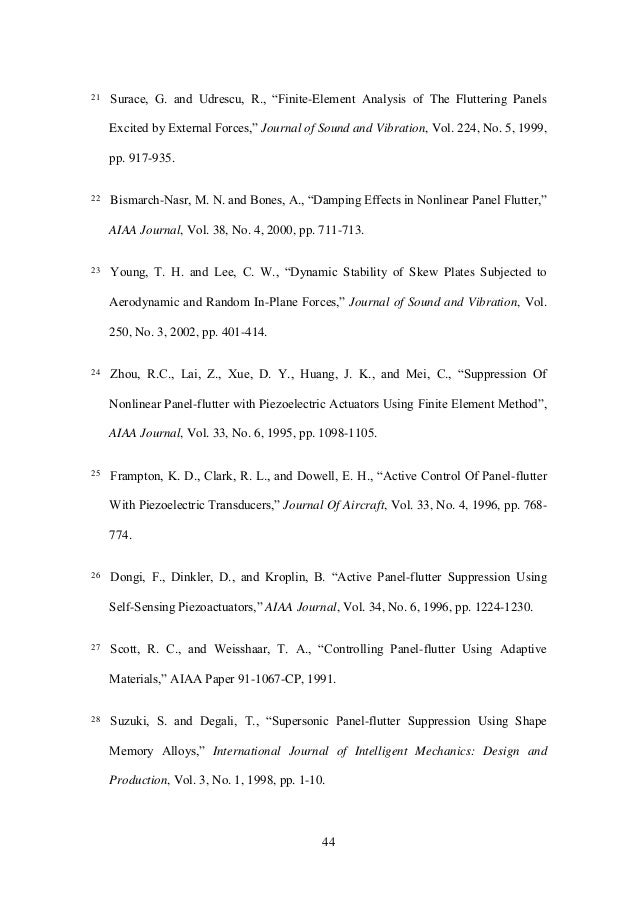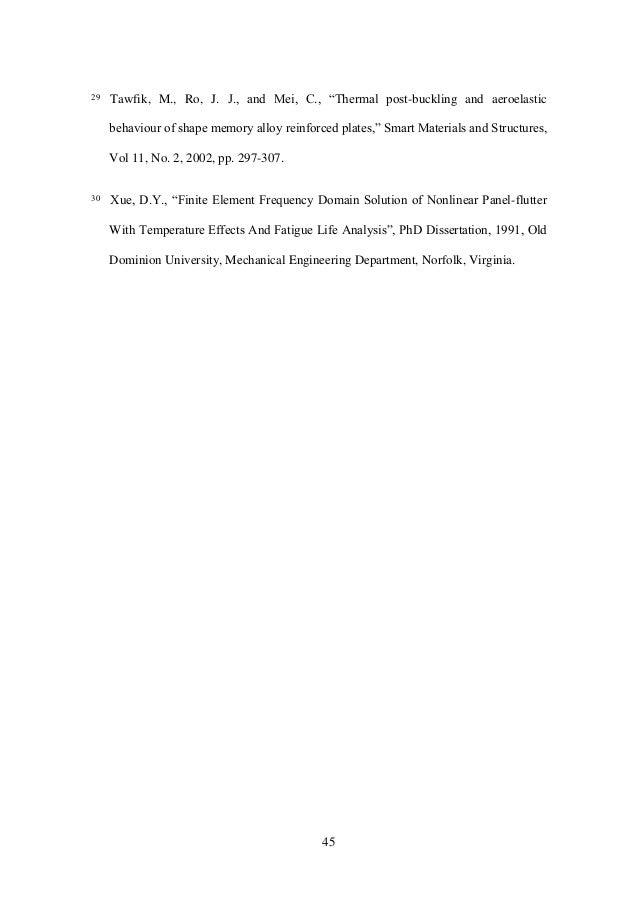Upcoming SlideShare
×

# Finite Element Analysis of the Aeroelasticity Plates Under Thermal and Aerodynamic Loading

At the flight condition of panel-flutter (usually supersonic flight conditions), the phenomenon is associated with elevated temperatures, produced from the aerodynamic heating through the boundary layer friction and the presence of shock waves. This heating adds to the complexity of the problem by introducing panel stiffness reduction and thermal loading, which might also be associated with post-buckling deflection. In the following, a literature review of panel-flutter analysis and control topics is presented.

#WikiCourses
https://wikicourses.wikispaces.com/TopicX+Nonlinear+Solid+Mechanics
https://eau-esa.wikispaces.com/Topic+Nonlinear+Solid+Mechanics

• Full Name
Comment goes here.

Are you sure you want to Yes No• Be the first to comment

### Finite Element Analysis of the Aeroelasticity Plates Under Thermal and Aerodynamic Loading

1. 1. Finite Element Analysis of the Aeroelasticity Plates Under Thermal and Aerodynamic Loading Mohammad Tawfik, PhD Aerospace Engineering Department Cairo University
2. 2. 2 Table of Contents 1. Introduction and Literature Review.....................................................................3 1.1. Panel-Flutter Analysis ....................................................................................5 1.2. Panel-Flutter Control......................................................................................9 2. Derivation of the Finite Element Model............................................................11 2.1. The Displacement Functions ........................................................................11 2.2. Displacement Function in terms of Nodal Displacement.............................12 2.3. Nonlinear Strain Displacement Relation......................................................15 2.4. Inplane Forces and Bending Moments in terms of Nodal Displacements ...18 2.5. Deriving the Element Matrices Using Principal of Virtual Work................19 2.5.1. Virtual work done by external forces .................................................23 3. Solution Procedures and Results of Panel Subjected to Thermal Loading .......25 4. Derivation of the Finite Element Model for Panel-flutter Problem...................28 4.1. Deriving the Element Matrices Using Principal of Virtual Works ..............28 4.2. 1st -Order Piston Theory ................................................................................29 5. Solution Procedure and Results of the Combined Aerodynamic-Thermal Loading Problem ..........................................................................................31 5.1. The Flutter Boundary ...................................................................................31 5.2. The Combined Loading Problem .................................................................34 5.3. The Limit Cycle Amplitude..........................................................................36 6. Concluding Remarks .........................................................................................40 References .............................................................................................................41
3. 3. 3 1. Introduction and Literature Review Panel-flutter is defined as the self-excited oscillation of the external skin of a flight vehicle when exposed to airflow along its surface ‎1 .‎As‎early‎as‎late‎1950’s,‎the‎problem‎ of panel-flutter has drawn‎the‎researchers’‎attention‎‎1 , but it has not been of high interest until the evolution of the National Aerospace Plane, the High Speed Civil Transport (HSCT), the Advanced Tactical Fighter projects, and F-22 fighter ‎2-‎4 among other projects of high-speed vehicles. At high speed maneuvering of flight vehicles, the external skin might undergo self- excited vibration due to the aerodynamic loading. This phenomenon is known as panel- flutter. Panel-flutter is characterized by having higher vibration amplitude in the third quarter length of the panel (Figure ‎1.1). This phenomena causes the skin panels of the flying vehicle to vibrate laterally with high amplitudes that cause high inplane oscillatory stresses; in turn, those stresses cause the panel to be a subject to fatigue failure. Figure ‎1.1 Sketch of panel-flutter phenomenon
4. 4. 4 It is thus required to determine the panel-flutter boundary (critical dynamic pressure) as well as the amplitude of vibration at which the panel will be oscillating in post-flutter conditions. Linear analysis of the panel structure could be used to predict the critical dynamic pressure of flutter, but is limited to that, as post flutter vibration is characterized by being of high amplitude which needs the application of nonlinear modeling techniques to describe. The linear analysis though, predicts exponential growth of the amplitude of vibration with the increase of the dynamic pressure in a post flutter condition. However, it is worth noting that under those conditions, the vibration of the panel will be influenced by inplane as well as bending stresses that lead to the limit cycle oscillation. Thus, failure of the panel does not occur at post-flutter dynamic pressures, but extended exposure to the panel-flutter decreases the fatigue life of the panel. One of the earliest reviews that covered the topic of panel flutter was that presented by Dowell in 1970. It covered the variety of available literature dealing with the problem. Analytical and numerical methods predicting the flutter boundary as well as the limit cycle amplitude for panels (beam models) were presented as well as the effect of presence of in-plane loading. Later, Bismarck-Nasr presented reviews of the finite element analysis of the aeroelastic problems of plates and shells. The study also included the cases of in-plane loading and its effects on the problem. Lately, Mei et al. presented another review that covered the topics of analytical and numerical analysis of panel flutter together with the topics involving the flutter control and delay.
5. 5. 5 Different methods were used to predict the post-flutter (limit cycle) attitude, which is a nonlinear phenomenon by nature, of the panel; modal transformation approach with direct numerical integration, harmonic balance, perturbation method, and nonlinear finite element method ‎1,‎9 were used for that purpose. The aerodynamic loading on the panel was also predicted using different approaches; unsteady supersonic potential flow ‎10 , linearized potential flow ‎1,‎4 , and quasi-steady piston theory. The most popular of which is the first order quasi-steady piston theory that was introduced by Ashley and Zartarian ‎11 . That approximate theory gives high accuracy results at high Mach numbers (M ∞>1.6). At the flight condition of panel-flutter (usually supersonic flight conditions), the phenomenon is associated with elevated temperatures, produced from the aerodynamic heating through the boundary layer friction and the presence of shock waves. This heating adds to the complexity of the problem by introducing panel stiffness reduction and thermal loading, which might also be associated with post-buckling deflection. In the following, a literature review of panel-flutter analysis and control topics is presented. 1.1. Panel-Flutter Analysis The non-linear finite element formulation, introduced by Mei ‎1 , was the basis on which Dixon and Mei ‎12 , Xue and Mei ‎3 , and Abdel-Motagalay et al. ‎13 , build their finite element models to analyze the flutter boundary, limit cycle, and the thermal problems, with the extension to random loading and SMA embedding introduced by Zhong ‎14 .
6. 6. 6 Different finite element models were developed to analyze the behavior of panels subject to aerodynamic loading. Mei ‎1 introduced the use of nonlinear finite element methods to predict the behavior of isotropic panels in the limit-cycle oscillations (LOC). In his work, he used the quasi-steady first order piston theory to predict the aerodynamic loading for M∞>1.6. He also presented a comparison of the effect of different structural boundary conditions on the critical dynamic pressure and on oscillation amplitude. Dixon and Mei ‎12 extended the use of finite element nonlinear analysis to composite panels. von Karman strain-displacement relations were used to represent the large deflections and the aerodynamic load was modeled using quasi- steady first-order piston theory. They solved the equations of motion using the linear- updated mode with a nonlinear time function (LUM/NTF) approximation. Results were also presented for different boundary conditions. Model enhancements were also developed to include the thermal effects as well as the flow direction. Xue and Mei ‎3 presented a very good study on the combined effect of aerodynamic forces and thermal loads on panel-flutter problems. They studied the effect of the temperature elevation on the critical dynamic pressure as well as the buckling temperature variation under different dynamic pressure conditions. The study also showed the effect of the different boundary conditions on the amplitude of the limit cycle oscillations (LOC). Abdel-Motagalay et al.‎13 studied the effect of flow direction on the panel-flutter behavior using first order shear deformation theory for laminated composite panels. They formulated the finite element nonlinear equations in structural node degrees of freedom, and then reduced the number of equations using a modal
7. 7. 7 transformation. The resulting reduced equations were then solved using the LUM/NTF approximation. Sarma and Varadan ‎9 adopted two methods to solve the nonlinear panel-flutter problem, the starting point of the first solution was calculated using nonlinear vibration mode and in the second they used linear mode as their starting point. They derived the equations from energy‎considerations‎using‎Lagrange’s‎equations‎of‎motion,‎and‎then‎ reduced the equations to nonlinear algebraic equations to solve a double eigenvalue problem. Frampton et al. ‎4,‎15 applied the linearized potential flow aerodynamics to the prediction and control of the flutter boundary using discrete infinite impulse response (IIR) filters with conventional ‎15 and modern ‎4 control theories. To get their solutions, they increased the non-dimensional dynamic pressure and calculated the coupled system eigenvalues until the point of coalescence at which the first complex eigenvalue appears. They studied the linear panel-flutter problem, thus, only presenting a prediction of the flutter boundary. Gray et al. ‎16 introduced the approximation of the third order unsteady piston theory aerodynamics for the flow over a 2-D panel. Both nonlinear aerodynamic and structure terms were considered in their finite element formulation. They also presented results for different support conditions. They concluded that the third order piston theory introduces a destabilizing effect as compared to the first order quasi-steady theory.
8. 8. 8 Benamar et al. ‎17,‎18 formulated the large amplitude plate vibration problem and developed the numerical model to apply the analysis to fully clamped plates. They claimed that the assumption of the space-time solution w(x,y,t) can be presented in the form w(x,y,t)=q(t)*f(x,y) may be inaccurate for nonlinear deflections. They suggested that for high amplitudes and low aspect ratios, the effect of nonlinear (plastic) material properties must be taken into consideration as well. They also presented a set of experimental results conducted to investigate the dynamic response characteristics of fully clamped plates at large vibration amplitudes ‎18 . Different aerodynamic models were introduced to the solution of the panel-flutter problem to enhance the results of the finite element model or introduce new ranges of analysis. Yang and Sung ‎10 introduced the unsteady aerodynamic model in their research on panel-flutter in low supersonic flow fields where the quasi-steady piston theory fails to produce accurate results. Liu et al. ‎19 introduced a new approach for the aerodynamic modeling of wings and panel in supersonic-hypersonic flight regimes. Their model was a generalization of the piston theory. Their model also accounts for the effects of wing thickness. Lately, studies of panel flutter were directed to enhancing the models and introducing different realistic parameters into the problem. Lee et al. modeled a panel with sheer deformable model as well as applying boundary conditions in the form a Timoshenko beams. Surace and Udrescu studied the panel flutter problem with higher order finite element model with the effect of external static pressure. While Bismarch- Nasr and Bones studied the effect of the aerodynamic damping on the panel flutter
9. 9. 9 attitude. Most recently, Young and Lee presented a study of the flutter of the plates subject to in-plane random loading. Those studies introduced more practical models for the problem of panel flutter which was and still is very hard to study experimentally. 1.2. Panel-Flutter Control Many researchers studied the problem of panel-flutter, but not as much studied the control of the flutter problem. The studies of the panel-flutter control were mostly conducted to increase the flutter boundaries (increase flutter Mach number). The main aim of controlling the panel-flutter is to increase the life of the panels subjected to fatigue stresses by delaying the flutter and/or decreasing the flutter amplitude. Different control algorithms as well as the application of smart material were studied to determine the feasibility of the application in the panel-flutter suppression problem. Zhou et al. ‎24 presented an optimal control design to actively suppress large- amplitude limit-cycle flutter motion of rectangular isotropic panels. They developed an optimal controller based on the linearized modal equations, and the norms of feedback control gain were employed to provide the optimal shape and position of the piezoelectric actuator. They concluded that the in-plane force induced by piezoelectric layers is insignificant in flutter suppression; on the other hand, the results obtained verified the effectiveness of the piezoelectric materials in the panel-flutter suppression especially for simply supported plates for which the critical dynamic pressure could be increased by about four times. Frampton et al. ‎15,‎25 presented a study of the effect of adding a self-sensing piezoelectric material to the panel, and using direct rate feedback. They concluded that the use of their scheme increased the flutter non-dimensional dynamic pressure significantly.
10. 10. 10 Dongi et al.‎26 used self-sensing piezoactuators as a part of a dynamic feedback control system to suppress flutter. They concluded that a linear observer-based-state feedback control system fails in the face of structural nonlinearity. They accomplished the required control using output feedback from a pair of collocated or self-sensing piezoactuators. They concluded that this technique possesses good robustness properties regarding nonlinearity, flight parameter variations, and pressure differentials. They also concluded that it has the advantage of being a simple feedback scheme that can be implemented with an analogue circuit. Scott and Weisshaar ‎27 introduced the use of adaptive material in the control of panel-flutter. They investigated the use of both piezoelectric material and Shape Memory Alloys in modifying the flutter characteristics of a simply supported panel. They concluded that for the piezoelectric material used in their study, no substantial improvement was accomplished; on the other hand, they concluded that the SMA had the potential for significantly increasing panel-flutter velocities, and that it is a possible solution for the panel-flutter problems associated with aerodynamic heating. The use of SMA in the delay of buckling and panel flutter was of interest to different studies. Suzuki and Degaki ‎28 investigated the use of SMA into the suppression of panel-flutter through the optimization of SMA thickness distribution into a 2-D panel. While Tawfik et al. presented a study that involved the plate model with combined thermal and aerodynamic loading. Their study extended to the investigation of partial embedding of SMA in the panels as well as a partial study of the effect of the fibers direction.
11. 11. 11 2. Derivation of the Finite Element Model In this section, the equation of motion with the consideration of large deflection are derived for a plate subject to external forces and thermal loading. The thermal loading is accounted for as a constant temperature distribution. The element used in this study is the rectangular 4-node Bogner-Fox-Schmidt (BFS) C1 conforming element (for the bending‎ DOF’s).‎ The‎ C1 type of elements conserves the continuity of all first derivatives between elements. 2.1. The Displacement Functions The displacement vector at each node for FE model is T vu yx w y w x w w             2 (‎2.1) The above displacement vector includes the membrane inplane displacement vector  T vu and transverse displacement vector T yx w y w x w w             2 . The 16-term polynomial for the transverse displacement function is assumed in the form 33 16 32 15 23 14 22 13 3 12 3 11 3 10 2 9 2 8 3 7 2 65 2 4321),( yxayxayxayxaxyayxa yaxyayxaxayaxyaxayaxaayxw   (‎2.2) or in matrix form      }{ xxyxyxyx1 3332232233322322 aH ayxyxyxyxxyyxyxyyw w  (‎2.3)
12. 12. 12 where  T aaaaaaaaaaaaaaaaa 16151413121110987654321}{  is the transverse displacement coefficient vector. In addition, the two 4-term polynomials for the inplane displacement functions can be written as xybybxbbyxu 4321),(  (‎2.4) xybybxbbyxv 8765),(  (‎2.5) or in matrix form      }{ 00001 bH bxyyxu u  and      }{ 10000 bH bxyyxv v  (‎2.6) where    T bbbbbbbbb 87654321 is the inplane displacement coefficient vector. The coordinates and connection order of a unit 4-node rectangular plate element are shown in Figure ‎2.1. Figure ‎2.1. Node Numbering Scheme 2.2. Displacement Function in terms of Nodal Displacement The transverse displacement vector at a node of the panel can be expressed by
13. 13. 13                                                      16 15 1 222222 2322322322 3232223222 3332232233322322 2 9664330220010000 3322332020100 3232302302010 1 a a a yxxyyxxyyxyx yxyxyxyxxyxyxyxyx yxxyyxxyyyxyxyxyx yxyxyxyxxyyxyxyyxxyxyxyx yx w y w x w w  (‎2.7) Substituting the nodal coordinates into equation (2.7), we obtain the nodal bending displacement vector {wb} in terms of {a} as follows,                                                                                                                                                                                                        16 15 14 13 12 11 10 9 8 7 6 5 4 3 2 1 2 2 32 32 222222 2322322322 3232223222 3332232233322322 2 32 2 32 4 2 4 4 4 3 2 3 3 3 2 2 2 2 2 1 2 1 1 1 0000300200010000 0000003000200100 0000000000010 0000000000001 9664330220010000 3322332020100 3232302302010 1 0000030020010000 0000000000100 0000000003002010 0000000000001 0000000000010000 0000000000000100 0000000000000010 0000000000000001 a a a a a a a a a a a a a a a a bb bb bbb bbb baabbaabbaba babababaababababa baabbaabbbabababa babababaabbababbaabababa aa aaa aa aaa yx w y w x w w yx w y w x w w yx w y w x w w yx w y w x w w (‎2.8) or     aTw bb  (‎2.9) From equation (2.9), we can obtain      bb wTa 1  (‎2.10) Substituting equation (2.10) into equation (2.3) then        bwbbw wNwTHw  1 (‎2.11) where the shape function for bending is
14. 14. 14      1  bww THN (‎2.12) Similarly, the inplane displacement {u, v} can be expressed by  b xyyx xyyx v u              10000 00001 (‎2.13) Substituting the nodal coordinates into the equation (2.13), we can obtain the inplane nodal displacement {wm} of the panel                                                                                8 7 6 5 4 3 2 1 4 4 3 3 2 2 1 1 0010000 0000001 10000 00001 0010000 0000001 00010000 00000001 b b b b b b b b b b abba abba a a v u v u v u v u (‎2.14) or     bTw mm  (‎2.15) From (2.15),      mm wTb 1  (‎2.16) Substituting equation (2.16) into equation (2.6) gives        mummu wNwTHu  1 (‎2.17)        mvmmv wNwTHv  1 (‎2.18) where the inplane shape functions are      1  muu THN (‎2.19)      1  mvv THN (‎2.20)
15. 15. 15 2.3. Nonlinear Strain Displacement Relation The von Karman large deflection strain-displacement relation for the deflections u, v, and w can be written as follows                                                                                                            yx w y w x w z y w x w y w x w x v y u y v x u xy y x 2 2 2 2 2 2 2 2 2 1 2 1    (‎2.21) or          zm  (‎2.22) where  m = membrane inplane linear strain vector,   = membrane inplane nonlinear strain vector,  z = bending strain vector. The inplane linear strain can be written in terms of the nodal displacements as follows               mmmmmm vu v u m wBwTCbCb x H y H y H x H x v y u y v x u                                                          1  (‎2.23) where
16. 16. 16                                          yx x y x H y H y H x H C vu v u m 010100 1000000 0000010 (‎2.24) and      1  mmm TCB (‎2.25) The inplane nonlinear strain can be written as follows                       bbb w w wBwTCaC a y H x H G y w x w x w y w y w x w     2 1 ][ 2 1 }{ 2 1 }{ 2 1 2 1 0 0 2 1 1                                                               (‎2.26) where the slope matrix and slope vector are                              x w y w y w x w 0 0  (‎2.27)                    y w x w G (‎2.28) and
17. 17. 17                           2322322322 3232223222 3322332020100 3232302302010 yxyxyxyxxyxyxyxyx yxxyyxxyyyxyxyxyx y H x H C w w  (‎2.29)      1  bTCB  (‎2.30) Combining equations (2.23) and (2.26), the inplane strain can be written as follows           bmmm ww   BB 2 1  (‎2.31) The strain due to bending can be written in terms of curvatures as follows          }{}{}{ 2 1 2 2 2 2 2 bbbbbb wBwTCaC yx w y w x w                                (‎2.32) where                                          222222 3232 3322 2 2 2 2 2 1812128660440020000 6622606200200000 6262060026002000 2 yxxyyxxyyxyx yxyxxxxyyx xyyxyyxyyx yx w y w x w Cb (‎2.33) and      1  bbb TCB (‎2.34) Thus, the nonlinear strain-nodal displacement relation can be written as                bbbmm m wzww z BBB       2 1 }{ (‎2.35)
18. 18. 18 2.4. Inplane Forces and Bending Moments in terms of Nodal Displacements In this section, the derivation of the relation presenting the inplane forces {N} and bending moments {M} in terms of nodal displacements for global equilibrium will be derived. Constitutive equation can be written in the form                           T T M N D A M N   0 0 (‎2.36) where ‎14 , QhA  extensional matrix (‎2.37) (a) Q h D 12 3  flexural matrix (c)     2/ 2/ ),,( h h T dzzyxTQN  inplane thermal loads (d)     2/ 2/ ),,( h h T zdzzyxTQM  thermal bending moment (e) and h thickness of the panel, {} thermal expansion coefficient vector, T(x,y,z) temperature increase distribution above the ambient temperature For constant temperature distribution in the Z-direction, the inplane and bending loading due to temperature can be written in the following form     2/ 2/ h h T dzQTN     0 2/ 2/   h h T zdzQTM  for isotropic plate. with
19. 19. 19               2 1 00 01 01 1 ][ 2     E Q (‎2.38) Expanding equation (2.36) gives                       Tm Tbmm Tm NNN NwAwA NAN            BB 2 1 ][ (‎2.39)     bb wDDM B][}]{[   (‎2.40) 2.5. Deriving the Element Matrices Using Principal of Virtual Work Principal of virtual work states that   0int  extWWW  (‎2.41) Virtual work done by internal stresses can be written as        V A TT ijij dAMNdVW }{}{int  (‎2.42) where                TTT b T m T m TT m T ww     BB   (‎2.43) and      T b T b T w B  (‎2.44) Note that      GG        2 1
20. 20. 20 Substituting equations (2.39), (2.40), (2.43), and (2.44) into equation (2.42), the virtual work done by internal stresses can be expressed as follows                                 dA wDw NwAwA ww W A bb T b T b Tbmm TTT b T m T m                                     BB BB BB ][ 2 1 * int       (‎2.45) The terms of the expansion of equation (2.45) are listed as follows        mm T m T m wAw BB (‎2.46) (a)         b T m T m wAw  BB 2 1 (b)      T T m T m Nw  B (c)          mm TTT b wAw BB   (d)             b TTT b wAw   BB 2 1 (e)        T TTT b Nw   B (f)       bb T b T b wDw BB ][ (g) Terms (a) and (g) of equation (2.46) can be written in the matrix form as               m b m b mb w w k k ww 0 0  (‎2.47) Where the linear stiffness matrices are    dADk A b T bb  BB ][][ (‎2.48)     dAAk A m T mm  BB][ (‎2.49)
21. 21. 21 While terms (b) + (d) of equation (2.46) can be written as                                                                                                                                                   A bm TT b mm TTT bb T m T m A mm TTT b mm TTT bb T m T m A mm TTT bb T m T m mbmbbmbmbnmb m b mb bmnm mb dA wBNw wAwwAw dA wAw wAwwAw dAwAwwAw wnwwnwwnw w w n nn ww             B BBBB BB BBBB BBBB 2 1 2 1 2 1 2 1 2 1 2 1 2 1 1 2 1 1 2 1 1 2 1 01 11 2 1 Note that                     bmmm ymxym xymxm xymym xymxm xym ym xm m T mm T wBNbCNGN y w x w NN NN N x w N y w N y w N x w N N N x w y w y w x w NwA                                                                                 0 0 B where      }{ mm xym ym xm m wA N N N N B            and          ymxym xymxm m NN NN N (‎2.50) Thus,       dAAnn A T m T bmmb   BB]1[]1[ (‎2.51)        dABNn A m T nm  B1 (‎2.52)
22. 22. 22 The first order nonlinear stiffness matrices,    nmmb nn 1&1 , are linearly dependent on the node DOF {wm}([Nm]) and {wb}([]). The second order nonlinear stiffness can be derived from term (e):                b TTT bb T b wAwwnw   BB 2 1 2 3 1  Thus,         dAAn A TT    BB 2 3 ]2[ (‎2.53) Also                                           bT TT bT TT bT TT b TyTxy TxyTxTT b TxyTy TxyTx TT b Txy Ty Tx TT bT TTT b wBNwbCNwGNw y w x w NN NN w N x w N y w N y w N x w w N N N x w y w y w x w wNw                                                                                              BBB BB BB 0 0 Thus,      dABNk A T T TN    B][ (‎2.54) where             TyTxy TxyTx T NN NN N (‎2.55) Term (f) of equation (2.46) can be written in matrix form as follows       dANp A T T mTm    B (‎2.56)
23. 23. 23 2.5.1. Virtual work done by external forces For the static problem, we may write:      A Surface iiext dAyxpw dSuTW ),(  (‎2.57) where T is the surface traction per unit area and p(x,y,t) is the external load vector. The right hand side of equation (2.57) can be rewritten as   b T b pw where     dAyxpNp A T wb  ),( (‎2.58) Finally, we may write                                                                m b mb bmnm TN m b W W N N NN K K K 00 02 3 1 01 11 2 1 00 0 0 0                  Tm b P P 0 0 (‎2.59) Equation (2.59) presents the static nonlinear deflection of a panel with thermal loading, which can be written in the form              TTN PPWNNKK         2 3 1 1 2 1 (‎2.60) Where  K is the linear stiffness matrix,  TNK  is the thermal geometric stiffness matrix,  1N is the first order nonlinear stiffness matrix,  2N is the second order nonlinear stiffness matrix,
24. 24. 24  P is the external load vector,  TP is the thermal load vector,
25. 25. 25 3. Solution Procedures and Results of Panel Subjected to Thermal Loading The solution of the thermal loading problem of the panel involves the solution of the thermal-buckling problem and the post-buckling deflection. In this chapter, the solution procedure for predicting the behavior of panel will be presented. For the case of constant temperature distribution, the linear part of equation 2.60 can be written as follows       0  WKTK TN (‎3.1) Which is an Eigenvalue problem in the critical temperature crT . Equation (2.60) that describes the nonlinear relation between the deflections and the applied loads can be also utilized for the solution of the post-buckling deflection. Recall            TTN PWNNKK         2 3 1 1 2 1 (2.60) Introducing the error function   W as follows                02 3 1 1 2 1         TTN PWNNKKW (‎3.2) which can be written using truncated Taylor expansion as follows          W dW Wd WWW    (‎3.3)
26. 26. 26 where              tan21 KNNKK dW Wd TN    (‎3.4) Thus, the iterative procedures for the determination of the post-buckling displacement can be expressed as follows               TiiiTNi PWNNKKW         2 3 1 1 2 1 (‎3.5)      iii WWK 1tan  (‎3.6)       ii WKW i    1 tan1 (‎3.7)       11   iii WWW  (‎3.8) Convergence occur in the above procedure, when the maximum value of the   1iW becomes less than a given tolerance tol ; i.e.   toliW  1max . Figure ‎3.1 presents the variation of the maximum transverse displacement of the panel when heated beyond the buckling temperature. Notice that the rate of increase of the buckling deformation is very high just after buckling, then it decreases as the temperature increases indicating the increase in stiffness due to the increasing influence of the nonlinear terms.
27. 27. 27 0 0.5 1 1.5 2 2.5 6 11 16 21 26 Temperature Increase (C) Wmax/Thickness Figure ‎3.1. Variation of maximum deflection of the plate with temperature increase.
28. 28. 28 4. Derivation of the Finite Element Model for Panel-flutter Problem In this section, the formulation presented in section 2 will be extended to include the dynamic (time dependent) terms. The aerodynamic formulation will be derived using first order quasi-steady piston theory which gives quite accurate aerodynamic model at high speed regimes. 4.1. Deriving the Element Matrices Using Principal of Virtual Works Extending the principle of virtual work to include the inertial terms, we may write,                                    A V iiext dA t v hv t u hutyxp t w hw dVuBW 2 2 2 2 2 2 ),,(   (‎4.1) where B is the body (inertial) forces per unit volume, T is the surface traction per unit area,  is the mass density per unit thickness, and h is the panel thickness. The first term of (4.1) can be used to derive the bending mass matrix from     bb T b wmw  , such that the bending mass matrix can be obtained by     dANNhm A w T wb  ][ (‎4.2) Similarly, the inplane mass matrix can be obtained by         dANNNNhm A v T vu T um   ][ (‎4.3) The equation of motions of the system can be written as
29. 29. 29                                                                              m b mb bmnmTN m b m b m b W WN N NNK K K W W M M 00 02 3 1 01 11 2 1 00 0 0 0 0 0           TmP 0 (‎4.4) 4.2. 1st -Order Piston Theory The fist order quasi-steady piston theory for supersonic flow, states that                                  x w a D t w a Dg x w t w vM Mq P a a 3 110 4 110 0 1 1 22   (‎4.5) where Pa is the aerodynamic loading, v the velocity of air-flow, M Mach number, q dynamic pressure =av2 /2, a air mass density,  12 M , ga non-dimensional aerodynamic damping   3 0 2 2   h Mva     non-dimensional aerodynamic pressure 110 3 2 D qa   o 2 1 4 110        ha D  D110 is the first entry of the laminate bending D(1,1) when all the fibers of the composite layers are aligned in the airflow x-direction. a is the panel length
30. 30. 30 The virtual work of the quasi-steady 1st -order piston theory aerodynamic loading is                      ba T bb aT b b w A T w T bbw a A T w T b A A a aext wa a wwg g w dAw x N a D NwdAwN a Dg Nw dA x w a D t w a Dg wdAwPW 3 0 3 110 4 110 0 3 110 4 110 0 . ][                                      (‎4.6) wheres the aerodynamic damping matrix [g] is defined by,          bb A w T w MM ha D dAN a D Ng 2 04 110 4 110     (‎4.7) and the aerodynamic influence matrix [aa] is defined by,     dA x N DNa w A T wa           110 (‎4.8) Finally, we get the equation of motion in the form                      )( 2 3 1 1 2 1 3 0 tPP WA a NNKKWG g WM T aTN a              (‎4.9) where                                               0 )( )( 00 0 00 0 0 0 tP tP A A G G M M M b a a m b
31. 31. 31 5. Solution Procedure and Results of the Combined Aerodynamic-Thermal Loading Problem In this section, the procedure of determining the critical non-dimensional dynamic pressure and the combined aerodynamic-thermal loading on the panel will be presented. The mass terms, as well as the time dependent terms, will be introduced to the equation of motion. The aerodynamic stiffness and damping terms are introduced by the quasi- steady piston theory. 5.1. The Flutter Boundary Recall from the previous chapter                      )( 2 3 1 1 2 1 3 0 tPP WA a NNKKWG g WM T aTN a              (4.9) Which can be reduced for the solution of the linear (pre-buckling and pre-flutter) problem to the following equation                    )(1 2 1 3 0 tPPWNA a KKWG g WM TaTN a            (‎5.1) Separating equations (5.1) into lateral and transverse directions, we obtain the following two equations                    )(1 2 1 3 0 tPPWNA a KK WG g WM bTbbnmaTNb b a bb           (‎5.2)
32. 32. 32 and        Tmmmmm PWKWM  (‎5.3) It can be assumed that the inplane mass term  mM is negligible ‎12 . Thus, equation (5.2) can be written in the form      Tmmm PKW    1 (‎5.4) Note that the terms related to N2 and N1mb are dropped as they depend on Wb which is essentially zero before buckling or flutter, while N1nm terms are kept as they depend in Wm which might have non-zero values depending on the boundary conditions. Substituting equation (5.4) into equation (5.2), we get                        )( 1 2 1 1 3 0 tPPPK WNA a KK WG g WM bTbTmm bnmaTNb b a bb                (‎5.5) Solving the homogeneous form of equation (5.5) reduces to                  01 2 1 3 0       bnmaTNbb a bb WNA a KKWG g WM    (‎5.6) Now, assuming the deflection function of the transverse displacement  bW to be in the form of     t bb ecW   (‎5.7) where  i is the complex panel motion parameter ( is the damping ratio and  is the frequency), c is the amplitude of vibration, and  b is the mode shape.
33. 33. 33 Substituting equation (5.7) into equation (5.6) we get,        0 t bb eKMc  (‎5.8) where    bob MM 2  ,  is the non-dimensional eigenvalue; given by o a o g            2 2 (‎5.9) and          nmaTNb NA a KKK 1 2 1 3    (‎5.10) From equation (5.8) we can write the generalized eigenvalue problem        0 bb KM (‎5.11) where  is the eigenvalue, and b is the mode shape, with the charactaristic equation written as,      0 KMb (‎5.12) Given that the values of are real for all values of  below the critical value, the iterative solution can be utilized to determine the critical non-dimensional dynamic pressure cr for temperatures less than the critical buckling temperature.
34. 34. 34 5.2. The Combined Loading Problem In this section, the procedures used to determine the buckling temperature under the influence of aerodynamic loading as well as the flutter boundary variation with elevated temperatures is presented. Recall the stiffness term from equation (5.10)          anmTNb A a NKKK 3 1 2 1    (5.10) This equation contains the combined effect of thermal and aerodynamic loading. In other words, the aerodynamic stiffness term (  aA a3  ) can be added to the procedure of section 3.2 to obtain the critical buckling temperature to investigate the effect of changing the dynamic pressure on the buckling temperature. Figure ‎5.1 presents the effect of changing the value dynamic pressure on the post- buckling deflection of the panel. It is seen clearly that the presence of flow increases the stiffness of the panel. 0 0.2 0.4 0.6 0.8 1 1.2 1.4 1.6 1.8 6 8 10 12 14 16 18 Temperature Increase (C) Wmax/Thickness Lamda=0 Lamda=150 Lamda=225
35. 35. 35 Figure ‎5.1. Variation of the maximum post-buckling deflection for different values of the dynamic pressure. Figure ‎5.2 presents a combined chart of the different regions of the combined loading‎ problem.‎ The‎ “Flat”‎ region‎ indicates‎ the‎ different‎ combinations‎ of‎ dynamic‎ pressure and temperature increase through which the panel will neither undergo flutter nor‎buckling.‎The‎“Buckled”‎region indicates the combination of dynamic pressure and temperature increase under which the panel undergoes static deflection due to buckling. Note that the curve indicating the change of the buckling temperature with dynamic pressure reaches an asymptotic value‎at‎dynamic‎pressure‎of‎242.‎The‎“Panel‎Flutter”‎ region indicates the combination of temperature and dynamic pressure under which the panel undergoes pure panel flutter; in other words, the panel becomes stiff enough, due to the aerodynamic pressure, that‎is‎does‎not‎suffer‎any‎buckling.‎Finally,‎the‎“Chaotic”‎ region indicates the combination of temperature increase and dynamic pressure under which the plate undergoes limit cycle flutter about a static deflection position due to thermal buckling. This final region is not going to be investigated in this project.
36. 36. 36 0 100 200 300 400 500 600 700 800 900 0 2 4 6 8 10 12 Temperature (C) DynamicPressure Flat Panel Buckled (Static) Panel Flutter (Dynamic) Chaotic Figure ‎5.2. The different regions of the combined loading problem. 5.3. The Limit Cycle Amplitude Following the same procedure outlined in the previous section with the only difference that we include all the nonlinear stiffness terms, we will end up with an equation similar to equation (5.8) in the form                       )(1 2 1 2 3 1 1 2 1 3 0 tPWN WA a NNKKWG g WM mbm banmTNbb a bb             (‎5.13)           Tmbmbmmmm PWNWKWM  1 2 1 (‎5.14) Ignoring the inertial terms of the membrane vibration, we may write,           bmbmTmmm WNKPKW 1 2 1 11     (‎5.15)
37. 37. 37 Substituting into the bending displacement equation we get,                               )(1 2 1 11 4 1 2 3 1 1 2 1 11 3 0 tPPKNWNKN WA a NNKKWG g WM Tmmbmbmbmbm banmTNbb a bb               (‎5.16) It can be shown that,         bmbmbmbnm WNKNWN 11 2 1 1 1  Which can be used to write the bending equation in its final form to be,                            )(1 2 1 2 3 1 11 2 1 1 1 3 0 tPPKN W NNKN A a KK WG g WM Tmmbm b mbmbm aTNb b a bb                        (‎5.17) Procedure similar to those described earlier can be used to write down the equation of motion in the form,        0 t bb eKMc  (‎5.18) Where,               2 3 1 11 2 1 1 3 NNKNA a KKK mbmbmaTNb     Since the nonlinear stiffness terms of the above equation depend on the amplitude of the vibration, an iterative scheme should be used. The following algorithm outlines the steps used in the iterative procedure. 1- Normalize the Eigenvector {}, obtained at the flutter point, using the maximum displacement.
38. 38. 38 2- Select a value for the amplitude c 3- Evaluate the linear and nonlinear stiffness terms. 4- Change the value of the nondimensional aerodynamic pressure . 5- Solve the eigenvalue problem for . 6- If coelacence occurs proceed, else goto step 4 7- Check the differences between the obtained eigenvector and the initial, if small, proceed, else normaize the eigenvector as described in step 1 and go to step 3. 8- The obtained dynamic pressure corresponds to the initially given amplitude 9- Go to step 2 It have to be noted that the above mentioned procedure is valid for the case when panel flutter occurs while the plate is not buckled or when the dynamic pressure is high enough that the buckled plate flat again; which does not cover the region of chaotic vibration. Figure ‎5.3 Presents and extended version of Figure ‎5.2. In this figure, we can notice the linear variaiotn of the dynamic pressure associated with different limit cycle amplitudes in the panel flutter region. Figure ‎5.4 presents a full map of the variation of both the limit cycle amplitude as well as the post-buckling deflection with the dynamic pressure for different values of the temperature increase. Note the distinction between the static and the dynamic regions.
39. 39. 39 0 200 400 600 800 1000 1200 0 2 4 6 8 10 Temperature (C) DynamicPressure Flutter Limit (C=0) C=1C=0.8C=0.5 Buckling Limit (Wmax=0) Figure ‎5.3. Variation of the dynamic pressure associated with different limit cycle amplitudes with temperature. 0 0.2 0.4 0.6 0.8 1 1.2 0 200 400 600 800 1000 1200 Non-Dimensional Dynamic Pressure Wmax/Thickness DT=0DT=3.4DT=6.4=TcrDT=9.4 DT=6.9 Buckling Flutter DT=9.4 DT=10.4 DT=7.4 DT=8.4 Figure ‎5.4. Variation of the limit cycle amplitude with dynamic pressure as well as the variation of the post-buckling amplitude with dynamic pressure for different values of the temperature increase.
40. 40. 40 6. Concluding Remarks This project report presents an extension of an earlier study that involved the post buckling deflection and the investigation of the effect of temperature on the flutter boundary of composite plate embedded with shape memory alloy fibers‎29 . The extension presented here is the investigation of the limit cycle vibration of the panel which is an essentially nonlinear dynamics problem. The other aspect that is not presented in this study is the investigation of the chaotic vibration region. This region requires the separation of the solution into two distinct values, namely the static deflection, which is due to the thermal loading, and the dynamic deflection, which is due to the flutter. The expansion involves extra nonlinear terms but is essentially straight forward from the point of stand of the current study‎30 .
41. 41. 41 References 1 Mei,‎C.,‎“A‎Finite‎Element‎Approach‎for‎Non-linear panel-flutter,”‎AIAA Journal, Vol. 15, No. 8, 1977, pp. 1107-1110. 2 Zhou,‎R.‎C.,‎Xue,‎D.‎Y.,‎and‎Mei,‎C.,‎“On‎Analysis‎of‎Nonlinear‎Panel-flutter at Supersonic‎ Speeds,”‎ Proceedings of the First Industry/Academy Symposium On Research For Future Supersonic And Hypersonic Vehicles, Vol. 1, Greensboro, North Carolina, 1994, pp. 343-348. 3 Xue,‎D.‎Y.,‎and‎Mei,‎C.,‎“Finite‎Element‎ Non-linear Panel-flutter with Arbitrary Temperature‎in‎Supersonic‎Flow,”‎AIAA Journal, Vol. 31, No. 1, 1993, pp. 154-162. 4 Frampton, K. D., Clark, R. L.,‎ and‎ Dowell,‎ E.‎ H.,‎ “State-Space Modeling For Aeroelastic‎Panels‎With‎Linearized‎Potential‎Flow‎Aerodynamic‎Loading,”‎Journal Of Aircraft, Vol. 33, No. 4, 1996, pp. 816-822. 5 Dowell,‎E.‎H.,‎“Panel‎Flutter:‎A‎Review‎of‎The‎Aeroelastic‎Stability‎of‎Plates and Shells,”‎AIAA Journal, Vol. 8, No. 3, 1970, pp. 385-399. 6 Bismarck-Nasr,‎ M.‎ N.,‎ “Finite‎ Element‎ analysis‎ of‎ Aeroelasticity‎ of‎ Plates‎ and‎ Shells,”‎Applied Mechanics Review, Vol. 45, No. 12, 1992, pp. 461-482. 7 Bismarck-Nasr,‎ M.‎ N.,‎ “Finite‎ Elements‎ in‎ Aeroelasticity‎ of‎ Plates‎ and‎ Shells,”‎ Applied Mechanics Review, Vol. 49, No. 10, 1996, pp. S17-S24.
42. 42. 42 8 Mei, C., Abdel-Motagaly,‎K.,‎and‎Chen,‎R.,‎“Review‎of‎Nonlinear‎Panel‎Flutter‎at‎ Supersonic‎and‎Hypersonic‎Speeds,”‎Applied Mechanics Review, Vol. 52, No. 10, 1999, pp. 321-332. 9 Sarma,‎ B.‎ S.,‎ and‎ Varadan,‎ T.‎ K.‎ “Non-linear Panel-flutter by Finite Element Method,”‎AIAA Journal, Vol. 26, No. 5, 1988, pp. 566-574. 10 Yang,‎T.‎Y.,‎and‎Sung,‎S.‎H.‎“Finite‎Element‎Panel-flutter in Three-Dimensional Supersonic Unsteady Potential‎ Flow,”‎ AIAA Journal, Vol. 15, No. 12, 1977, pp. 1677-1683. 11 Ashley,‎H.,‎and‎Zartarian,‎G.,‎“Piston‎Theory‎– A New Aerodynamic Tool for the Aeroelastician,”‎Journal of Aeronautical Sciences, Vol. 23, No. 12, 1956, pp. 1109- 1118. 12 Dixon, I. R., and Mei,‎ C.,‎ “Finite‎ Element‎ Analysis‎ of‎ Large-Amplitude Panel- flutter‎of‎Thin‎Laminates,”‎AIAA Journal, Vol. 31, No. 4, 1993, pp. 701-707. 13 Abdel-Motagaly,‎K.,‎Chen,‎R.,‎and‎Mei,‎C.‎“Nonlinear‎Flutter‎of‎Composite‎Panels‎ Under Yawed Supersonic Flow Using Finite Elements,”‎AIAA Journal, Vol. 37, No 9, 1999, pp. 1025-1032. 14 Zhong,‎Z.‎“Reduction‎of‎Thermal‎Deflection‎And‎Random‎Response‎Of‎Composite‎ Structures‎‎With‎Embedded‎Shape‎memory‎Alloy‎At‎Elevated‎Temperature”,‎PhD‎ Dissertation, 1998, Old Dominion University, Aerospace Department, Norfolk, Virginia.
43. 43. 43 15 Frampton,‎Kenneth‎D.,‎Clark,‎Robert‎L.,‎and‎Dowell,‎Earl‎H.‎“Active‎Control‎Of‎ Panel-flutter‎With‎Linearized‎Potential‎Flow‎Aerodynamics”,‎AIAA‎Paper‎95-1079- CP, February 1995. 16 Gray, C. E., Mei, C., and Shore, C. P.,‎“Finite‎Element‎Method‎for‎Large-Amplitude Two-Dimensional Panel-flutter‎at‎Hypersonic‎Speeds,”‎AIAA Journal, Vol. 29, No. 2, 1991, pp. 290-298. 17 Benamar,‎R,‎Bennouna,‎M.‎M.‎K.,‎and‎White‎R.‎G.‎“The‎effect‎of‎large‎vibration‎ amplitudes on the mode shapes and natural frequencies of thin elastic structures PART‎ II:‎ Fully‎ Clamped‎ Rectangular‎ Isotropic‎ Plates”,‎ Journal of Sound and Vibration, Vol. 164, No. 2, 1993, pp. 295-316. 18 Benamar,‎R,‎Bennouna,‎M.‎M.‎K.,‎and‎White‎R.‎G.‎“The‎effect‎of‎large‎vibration‎ amplitudes on the mode shapes and natural frequencies of thin elastic structures PARTIII: Fully Clamped Rectangular Isotropic Plates – Measurements of The Mode Shape Amplitude Dependence And The Spatial Distribution Of Harmonic Distortion”,‎Journal of Sound and Vibration, Vol. 175, No. 3, 1994, pp. 377-395. 19 Liu,‎ D.‎ D.,‎ Yao,‎ Z.‎ X.,‎ Sarhaddi,‎ D.,‎ and‎ Chavez,‎ F.,‎ “From‎ Piston‎ Theory‎ to‎ Uniform Hypersonic-Supersonic‎Lifting‎Surface‎Method,”‎Journal of Aircraft, Vol. 34, No. 3, 1997, pp. 304-312. 20 Lee, I., Lee, D.-M., and Oh, I.-K,‎ “Supersonic‎ Flutter‎ Analysis‎ of‎ Stiffened‎ Laminated‎Plates‎Subject‎to‎Thermal‎Load,”‎Journal of Sound and Vibration, Vol. 224, No. 1, 1999, pp. 49-67.
44. 44. 44 21 Surace,‎ G.‎ and‎ Udrescu,‎ R.,‎ “Finite-Element Analysis of The Fluttering Panels Excited by‎External‎Forces,”‎Journal of Sound and Vibration, Vol. 224, No. 5, 1999, pp. 917-935. 22 Bismarch-Nasr,‎M.‎N.‎and‎Bones,‎A.,‎“Damping‎Effects‎in‎Nonlinear‎Panel‎Flutter,”‎ AIAA Journal, Vol. 38, No. 4, 2000, pp. 711-713. 23 Young,‎ T.‎ H.‎ and‎ Lee,‎ C.‎ W.,‎ “Dynamic Stability of Skew Plates Subjected to Aerodynamic and Random In-Plane‎Forces,”‎Journal of Sound and Vibration, Vol. 250, No. 3, 2002, pp. 401-414. 24 Zhou,‎ R.C.,‎ Lai,‎ Z.,‎ Xue,‎ D.‎ Y.,‎ Huang,‎ J.‎ K.,‎ and‎ Mei,‎ C.,‎ “Suppression‎ Of‎ Nonlinear Panel-flutter with Piezoelectric‎Actuators‎Using‎Finite‎Element‎Method”,‎ AIAA Journal, Vol. 33, No. 6, 1995, pp. 1098-1105. 25 Frampton,‎K.‎D.,‎Clark,‎R.‎L.,‎and‎Dowell,‎E.‎H.,‎“Active‎Control‎Of‎Panel-flutter With‎Piezoelectric‎Transducers,”‎Journal Of Aircraft, Vol. 33, No. 4, 1996, pp. 768- 774. 26 Dongi,‎ F.,‎ Dinkler,‎ D.,‎ and‎ Kroplin,‎ B.‎ “Active‎ Panel-flutter Suppression Using Self-Sensing‎Piezoactuators,”‎AIAA Journal, Vol. 34, No. 6, 1996, pp. 1224-1230. 27 Scott,‎ R.‎ C.,‎ and‎ Weisshaar,‎ T.‎ A.,‎ “Controlling‎ Panel-flutter Using Adaptive Materials,”‎AIAA‎Paper‎91-1067-CP, 1991. 28 Suzuki,‎ S.‎ and‎ Degali,‎ T.,‎ “Supersonic‎ Panel-flutter Suppression Using Shape Memory‎ Alloys,”‎ International Journal of Intelligent Mechanics: Design and Production, Vol. 3, No. 1, 1998, pp. 1-10.
45. 45. 45 29 Tawfik, M., Ro, J. J.,‎ and‎ Mei,‎ C.,‎ “Thermal‎ post-buckling and aeroelastic behaviour‎of‎shape‎memory‎alloy‎reinforced‎plates,”‎Smart‎Materials‎and‎Structures,‎ Vol 11, No. 2, 2002, pp. 297-307. 30 Xue,‎D.Y.,‎“Finite‎Element‎Frequency‎Domain‎Solution‎of‎Nonlinear‎Panel-flutter With‎Temperature‎Effects‎And‎Fatigue‎Life‎Analysis”,‎PhD‎Dissertation,‎1991,‎Old‎ Dominion University, Mechanical Engineering Department, Norfolk, Virginia.

### Be the first to comment

•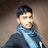#### tamilaghil

Jul. 26, 2015
•#### shvdubai

Jan. 29, 2016
•#### ssuser387981

Mar. 16, 2017
•#### NavyaShree70

Apr. 22, 2019

Total views

1,871

On Slideshare

0

From embeds

0

Number of embeds

221

58

Shares

0